Jekyll2023-08-17T11:54:50+02:00http://www.pokutta.com/blog/feed.xmlOne trivial observation at a timeEverything Mathematics, Optimization, Machine Learning, and Artificial IntelligenceAccelerated and Sparse Algorithms for Approximate Personalized PageRank and Beyond2023-08-17T01:00:00+02:002023-08-17T01:00:00+02:00http://www.pokutta.com/blog/research/2023/08/17/aapprTL;DR: This is an informal discussion of our recent paper Accelerated and Sparse Algorithms for Approximate Personalized PageRank and Beyond by David Martinez-Rubio, Elias Wirth, and Sebastian Pokutta. We answer the open question to the COLT 2022 open problem by Fountoulakis and Yang [FY] in the affirmative, presenting sparse accelerated optimization algorithms for the $\ell_1$-regularized personalized PageRank problem.

Written by Elias Wirth.

### Introduction

With the rise of large-scale networks, the study of local graph clustering has gained significant attention, fueling extensive research into local graph clustering algorithms [FY, FRS]. When graphs become large in the number of vertices and edges, practitioners run into memory issues. Thus, local algorithms operate only on a local subset of vertices and edges of the graph. At the heart of local graph clustering is the approximate personalized PageRank algorithm (APPR) [ACL], which offers an approximation to the solution of the PageRank linear system [PBM] within an undirected graph. This technique rounds the approximate solution to reveal local partitions within the graph’s structure.

Although the output of APPR doesn’t inherently present itself as the solution to an optimization problem, recent advancements by Fountoulakis et al. [FRS] introduced a variational formulation of local graph clustering as an $\ell_1$-regularized convex optimization problem, which Fountoulakis et al. solved using the iterative shrinkage-thresholding algorithm (ISTA) [PB]. Subsequently, Fountoulakis and Yang [FY] then raised the question whether the variational formulation could be addressed with accelerated methods whose per iteration complexity does not depend on the size of the whole graph, but on the size of local objects like the support of solution or its set of neighbors, the fast iterative shrinkage-threshholding algorithm (FISTA) [PB]. We answer this question in the affirmative by presenting several accelerated methods.

### Preliminaries

We briefly recall the problem formulation. We are given an undirected graph $G = (V, E)$, where $V$ and $E$ are the vertex and edge set, respectively. Let $n$ denote the number of vertices. Let $A$, $D$, and $L = I - D^{-1/2}A D^{-1/2}$ denote the associated adjacency, degree, and Laplacian matrices, respectively. Given a parameter $\alpha \in ]0, 1[$, we further define the positive definite matrix

\begin{align*} Q = \alpha I + \frac{1-\alpha}{2}L, \end{align*}

which satisfies $\alpha I \preccurlyeq Q \preccurlyeq 2 I$. Then, the variational formulation derived in [FRS] boils down to solving the constrained convex optimization problem

\begin{align*} \tag{OPT} \min_{x\in\mathbb{R}_{\geq 0}} g(x), \end{align*}

where

\begin{align*} g(x) = \frac{1}{2} \langle x, Qx\rangle + \alpha \langle -D^{-1/2}s + \rho D^{1/2}\mathbf{1}, x\rangle \end{align*}

and $s\in\Delta_n$ is a probability distribution over the vertices of $G$ called teleportation distribution and $\rho\in [0, 1]$ is the regularization parameter.

Let

\begin{align*} x^\esx := \text{argmin}_{x\in\mathbb{R}_{\geq 0}} g(x) \end{align*}

denote the optimal solution with support $$S^\esx := \text{supp}(x^\esx)$$. Note that for our setting, we generally have $$\lvert S^\esx \rvert << n$$, that is, the optimizer is highly sparse.

Finally, for a subset of vertices $S \subseteq V$, we define the volume of $S$ as $$\text{vol}(S) := \sum_{i \in S} d_i + \lvert S \rvert$$, that is, as the sum of the degrees of vertices in $S$, plus $$\lvert S \rvert$$ and the internal volume of $S$ as $\tilde{\text{vol}}(S) := \lvert S \rvert + \sum_{(i,j) \in E} \mathbf{1}_{\lbrace i,j \in S\rbrace}$, that is, as the sum of edges of the subgraph induced by $S$, plus $\lvert S \rvert$.

### Contributions

We extend the geometrical observations from [FRS] to derive four new algorithms for (OPT), all of which are accelerated in the sense that their time complexities are either completely independent of the condition number $$\frac{1}{\alpha}$$ or improve the dependence to $$\frac{1}{\sqrt{\alpha}}$$ compared to ISTA’s time complexity of order $$\tilde{O}(\text{vol}(S^\esx \frac{1}{\alpha})$$. We thus answer the open question in [FY] in the affirmative, providing multiple accelerated algorithms for (OPT). Note that all our algorithms are local, that is, generally don’t access the full graph and consequently, the per-iteration cost is not in $$O(n)$$ but depends on $$O(\abs{N(S^\esx)})$$ instead, where $$N(S^\esx)$$ denotes the neighbourhood of $$S^\esx$$.

Algorithm Time complexity Space complexity
ISTA [FRS] $$\tilde{O}(\text{vol}(S^\esx) \frac{1}{\alpha})$$ $$O(\lvert S^\esx \rvert )$$
Conjugate directions PageRank algorithm (CDPR) $$O(\lvert S^\esx \rvert^3 + \lvert S^\esx \rvert \text{vol}(S^\esx))$$ $$O(\lvert S^\esx \rvert^2 )$$
Accelerated sparse PageRank (ASPR) $$\tilde{O}(\lvert S^\esx \rvert \tilde{\text{vol}}( S^\esx ) \frac{1}{\sqrt{\alpha}} + \lvert S^\esx \rvert \text{vol}(S^\esx))$$ $$O(\lvert S^\esx \rvert )$$
Conjugate-gradients ASPR (CASPR) $$\tilde{O}(\lvert S^\esx \rvert \tilde{\text{vol}}( S^\esx ) \min\lbrace \frac{1}{\sqrt{\alpha}}, \lvert S^\esx \rvert \rbrace + \lvert S^\esx \rvert \text{vol}(S^\esx))$$ $$O(\lvert S^\esx \rvert )$$
Laplacian-solver ASPR (LASPR) $$\tilde{O}(\lvert S^\esx \rvert \tilde{\text{vol}}( S^\esx )) + O( \lvert S^\esx \rvert \text{vol}( S^\esx ))$$ $$O(\lvert S^\esx \rvert )$$

Our contribution extends beyond theory, as we offer complete julia implementations of all algorithms (excluding LASPR due to numerical instability) on GitHub. In our numerical experiments, we find that CASPR remarkably outperforms other algorithms in terms of solution sparsity and runtime efficiency. We compare the performance of all algorithms on the patent_cit_us [LK] graph consisting of 3,774,768 vertices and 16,518,948 edges. In the paper, we compare the methods on several other graphs, but the results are similar across problem instances. The parameters are $\alpha=0.01$ and $\rho=0.0001$ and the algorithms are run up to accuracy $\epsilon=10^{-6}$.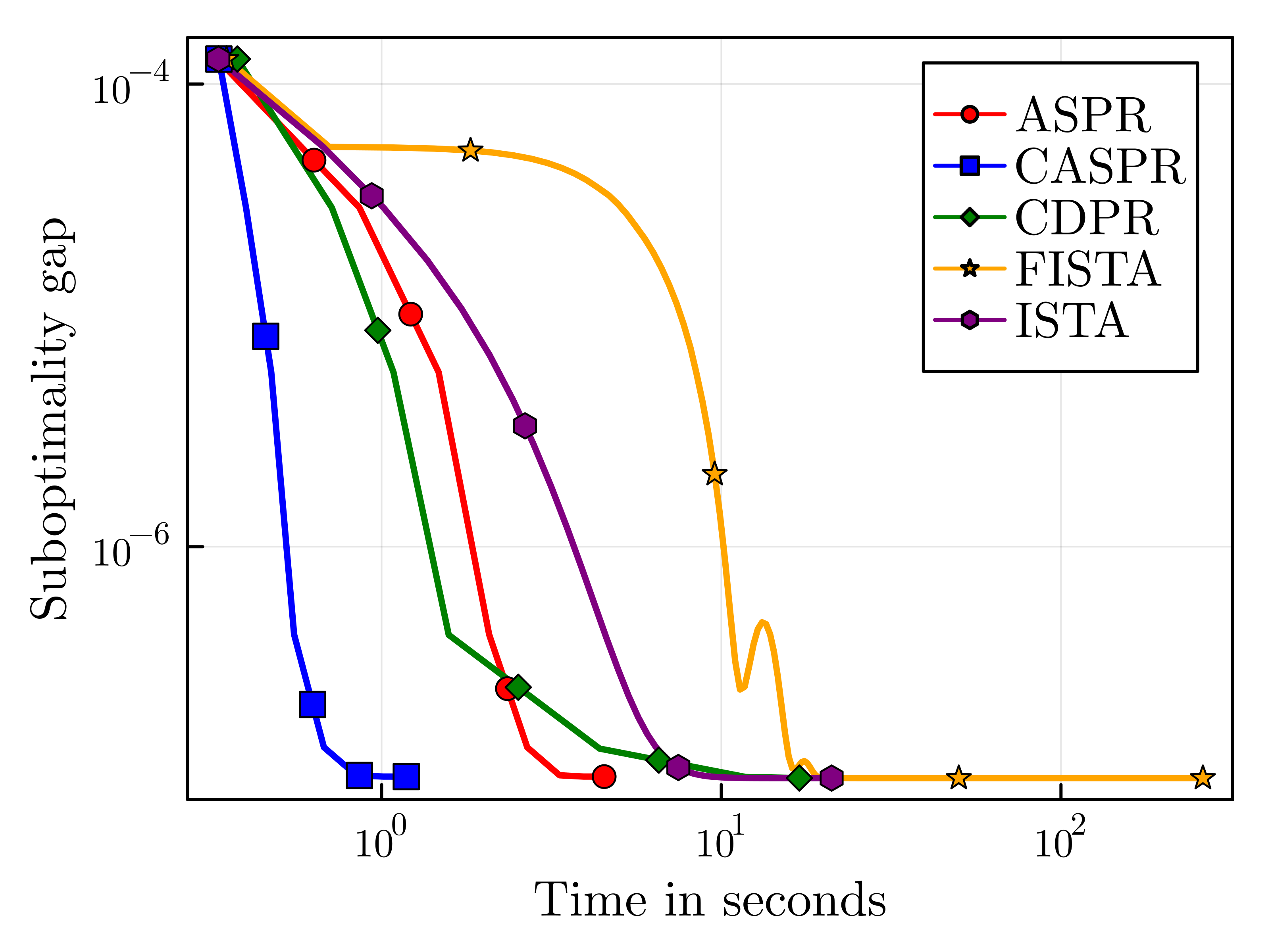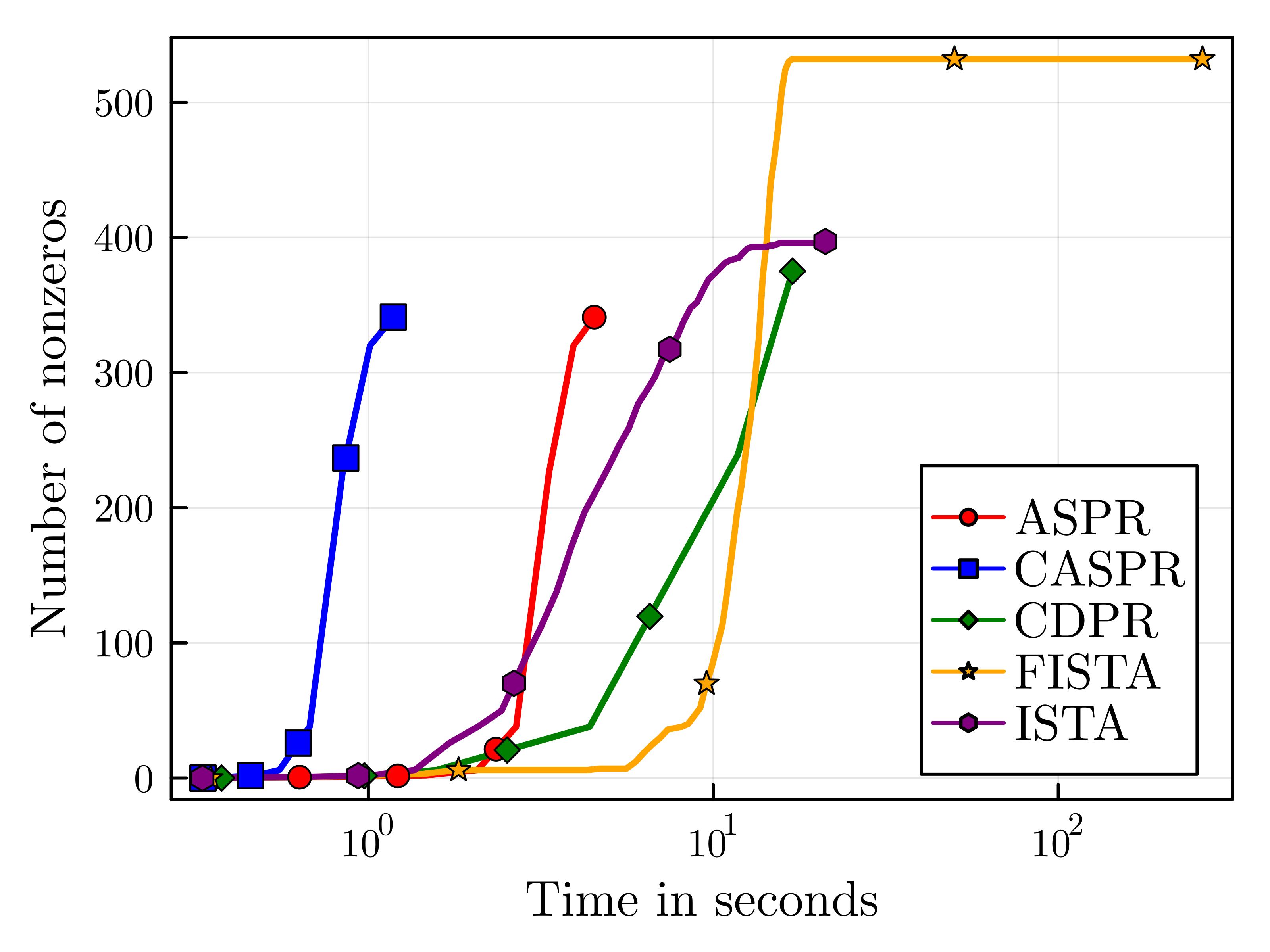Figure 1. Running time comparison (left) and sparsity comparison (right)

[ACL] Reid Andersen, Fan R. K. Chung, and Kevin J. Lang. “Local Graph Partitioning using PageRank Vectors”. In: 47th Annual IEEE Symposium on Foundations of Computer Science (FOCS 2006), 21-24 October 2006, Berkeley, California, USA, Proceedings. IEEE Computer Society, 2006, pp. 475–486.

[FRS] Kimon Fountoulakis, Farbod Roosta-Khorasani, Julian Shun, Xiang Cheng, and Michael W Mahoney. “Variational perspective on local graph clustering”. In: Mathematical Programming 174.1 (2019), pp. 553–573.

[FY] Kimon Fountoulakis and Shenghao Yang. “Open Problem: Running time complexity of accelerated ℓ1-regularized PageRank”. In: Proceedings of the Conference on Learning Theory. PMLR. 2022, pp. 5630–5632.

[LK] Jure Leskovec and Andrej Krevl. SNAP Datasets: Stanford Large Network Dataset Collection. http:// snap.stanford.edu/ data. June 2014.

[PB] Neal Parikh and Stephen P. Boyd. “Proximal Algorithms”. In: Found. Trends Optim. 1.3 (2014), pp. 127–239.

[PBM] Lawrence Page, Sergey Brin, Rajeev Motwani, and Terry Winograd. The PageRank Citation Ranking: Bringing Order to the Web. Technical Report 1999-66. Stanford InfoLab, Nov. 1999.

]]>
Sparse Model Soups: A Recipe for Improved Pruning via Model Averaging2023-08-05T01:00:00+02:002023-08-05T01:00:00+02:00http://www.pokutta.com/blog/research/2023/08/05/abstract-SMSTL;DR: This is an informal summary of our recent paper Sparse Model Soups: A Recipe for Improved Pruning via Model Averaging by Max Zimmer, Christoph Spiegel, and Sebastian Pokutta. Recent work [WM] demonstrated that generalization performance can be significantly improved by merging multiple models fine-tuned from the same base model. However, averaging sparse models leads to a decrease in overall sparsity, due to different sparse connectivities. We discovered that by exploring a single retraining phase of Iterative Magnitude Pruning (IMP) [HPTD] with various hyperparameters, we can create models suitable for averaging while retaining the same sparse connectivity from the pruned base model. Building on this idea, we introduce Sparse Model Soups (SMS), an IMP-variant where every prune-retrain cycle starts with the averaged model from the previous phase. SMS not only maintains the sparsity pattern but also offers full modularity and parallelizability, and substantially enhances the performance of IMP.

Written by Max Zimmer.

### Introduction

The field of Neural Networks has witnessed an explosion of interest over the past decade, revolutionizing numerous research and application areas by pushing the boundaries of feasibility. However, the widespread adoption of these networks is not without its challenges: Larger networks are notorious for their long training durations, extensive storage requirements, and consequently, high financial and environmental costs. Pruning, or the act of eliminating redundant parameters, serves as a valuable countermeasure to these issues. It facilitates the creation of sparse models that significantly reduce storage and floating-point operation (FLOP) demands, all while maintaining performance levels akin to their dense counterparts. A classic approach to achieve this is through Iterative Magnitude Pruning (IMP, [HPTD]), which initially trains a dense model from scratch and then iteratively prunes a fraction of the weights with smallest magnitude followed by a retraining phase to recover pruning-induced performance degradation. For a more comprehensive understanding of pruning and particularly IMP, we recommend our blogpost discussing our ICLR2023 paper How I Learned to Stop Worrying and Love Retraining.

On the other hand, diverse strategies exist that aim to enhance model performance by leveraging the combined strengths of multiple models. This ‘wisdom-of-the-crowd’ approach is typically seen in the form of prediction ensembles, where the predictions of $$m$$ distinct models are collectively used to form a single, consolidated prediction. However, this method comes with a significant drawback: it requires each of the $$m$$ models to be evaluated to derive a single prediction. To circumvent this efficiency hurdle, Model Soups [WM] build a single model by averaging the parameters of the $$m$$ networks. This results in an inference cost that is asymptotically constant with respect to $$m$$, significantly improving efficiency.

Yet, the concept of averaging multiple models isn’t without its disadvantages when contrasted with prediction ensembles. While prediction ensembles thrive on model diversity, model soups necessitate a considerable degree of similarity in the weight space. Averaging two models that are vastly dissimilar can lead the resultant model soup to land in an area of the weight space characterized by much higher loss. In fact, if two models are trained from scratch with only different random seeds, the average of their parameters will likely underperform compared to the individual models. Wortsman et al. [WM] showcase a method to create models suitable for averaging: by fine-tuning $$m$$ distinct copies from a shared pre-trained base model. This approach ensures the required similarity in the weight space to create effective model soups.

A less prominent problem is that of combining multiple sparse models. Consider $$m$$ different sparse models. In general, these models will have different sparse connectivities and averaging their parameters will cancel out most of the zero entries, effectively reducing the sparsity of the models and requiring to re-prune at the expense of accuracy. Figure 1 below illustrates this phenomenon.Figure 1. Constructing the average (middle) of two networks with different sparsity pattern (left, right) can reduce the overall sparsity level, turning pruned weights (dashed) into non-zero ones (solid). Weights reactivated in the averaged model are highlighted in orange.

In this work, we address the challenge of generating models that can be averaged without disrupting their sparsity patterns. Central to our approach is the finding that conducting a single prune-retrain phase with varied hyperparameter settings such as random seed, weight decay, etc., yields models that are a) suitable for averaging, and b) retain the same sparse connectivity inherited from the original pruned base model. Building on this insight, we introduce Sparse Model Soups (SMS), a variant of IMP that commences each phase with an averaged model from the prior phase. This approach not only preserves sparse connectivity throughout the entire sparsification process, but also significantly enhances the performance of IMP.

### Merging Sparse Models after One Shot pruning and Retraining

In most cases, averaging arbitrary sparse models will inadvertently reduce the sparsity level of the resulting model compared to the individual ones, potentially also compromising accuracy as these models might not be suited for averaging. However, the scenario changes when we examine models derived from a shared parent model, a technique prevalent in transfer learning [WM].

Our exploration reveals that it’s indeed feasible to average multiple models that are retrained from the same pruned base model. Here’s our approach: Consider the initial phase of the IMP process. We start with a pretrained model, prune it to achieve a desired level of sparsity, and then retrain it to compensate for the losses induced by pruning. Instead of merely retraining once, we create $$m$$ copies of this pruned model and individually retrain each one under a unique hyperparameter configuration. This could involve changing factors such as the strength of weight decay or the random seed which influences the batch ordering.

When we average two (or more) models, there’s no reduction in sparsity since all models stem from the same pruned base model. Interestingly, these models are also amenable to averaging. To illustrate this, consider Figure 2 (below) where we pruned ResNet-50, pretrained on ImageNet, to 70% sparsity. We then examined all $$m \choose 2$$ combinations of retrained models, plotting their maximum test accuracy on the x-axis against the test accuracy of their average on the y-axis. The results clearly show net improvements for nearly all configurations. Interestingly, merely changing the random seed led to consistent and significant performance gains of up to 1%, despite the fact that we’re averaging just two models.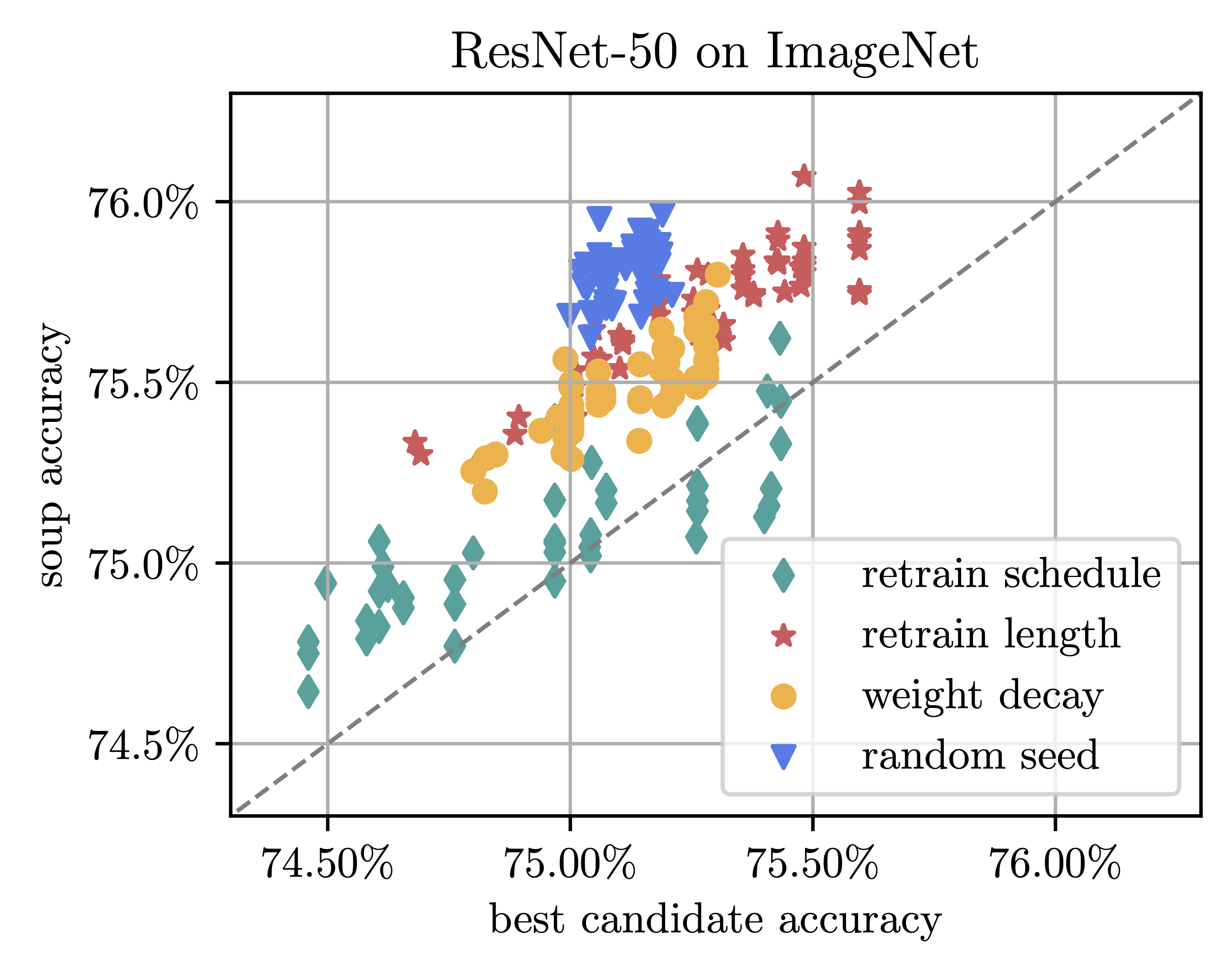Figure 2. Accuracy of average of two models vs. the maximal individual accuracy. All models are pruned to 70% sparsity (One Shot) and retrained, varying the indicated hyperparameters.

### SMS: Sparse Model Soups

Having established that the initial phase of IMP is suitable to training $$m$$ models in parallel and averaging them, we’re faced with another intriguing question: Is it possible to maintain the overall sparsity while averaging models after multiple phases? Surprisingly, the answer is negative. Although models are averageable after a single prune-retrain cycle, another cycle of pruning and retraining results in models with divergent sparsity patterns: they share a common pattern after the first phase, but individual pruning leads to distinctive sparse connectivities among them.

This issue brings us to our proposed solution, the Sparse Model Soups (SMS) algorithm, as illustrated below. By capitalizing on the modularity of IMP’s phases, we construct the average model after each phase, merging $$m$$ retrained models into a single model. This entity then serves as the starting point for the subsequent phase, guaranteeing that the sparsity patterns are consistent across all individual models.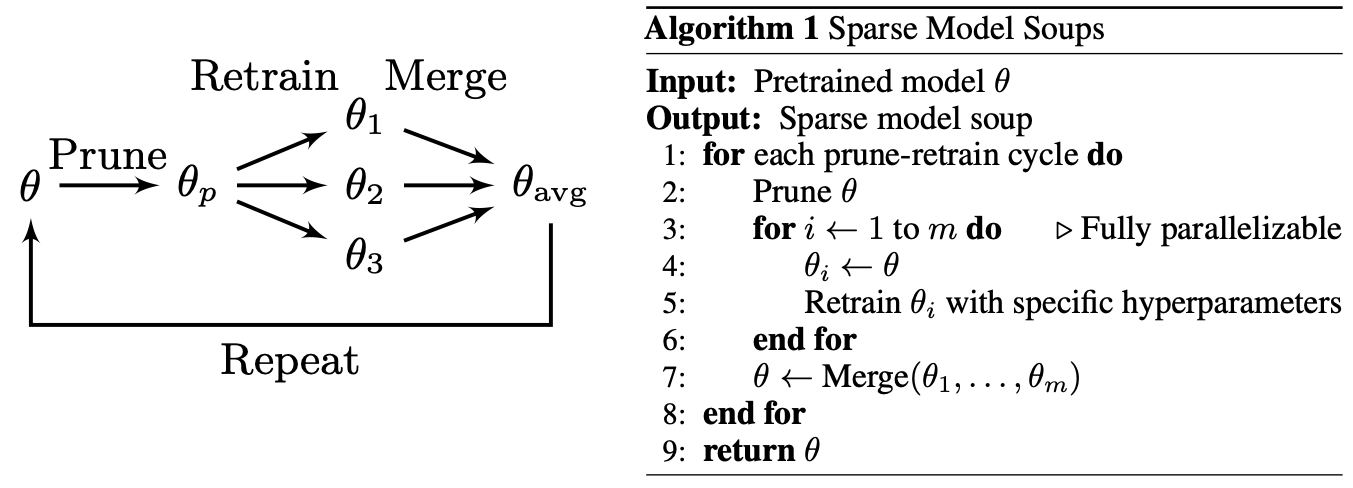Figure 4. Left: Sketch of the algorithm for a single phase and $$m=3$$. Right: Pseudocode for SMS. Merge$$(\cdot)$$ takes $$m$$ models as input and returns a linear combination of the models.

We now evaluate SMS against several critical baselines, comparing it at each prune-retrain phase with the top-performing single model amongst all candidates for averaging (best candidate). In addition, we also contrast it against the mean candidate, standard IMP (i.e., when $$m=1$$), and an enhanced variant of IMP that is retrained $$m$$ times longer, which we denote as IMP+. Furthermore, we compare SMS with a method that executes standard IMP $$m$$ times, then averages the models post-final phase, a strategy we call IMP-AVG. This method seeks to mitigate potential reduction in sparsity by re-pruning the model [YIN].

Table 1 outlines the results for three-phase IMP, using WideResNet-20 on CIFAR-100 and ResNet-50 on ImageNet. The left column represents the baselines, while the three central columns correspond to phases and sparsity levels, targeting 98% sparsity for CIFAR-100 and 90% for ImageNet. Each central column further breaks down into three sub-columns, indicating the number of models to average (3, 5, or 10). We observe that SMS consistently outperforms the test accuracy of the best candidate, often by a margin of 1% or more. This illustrates that the models are indeed amenable to averaging after retraining, resulting in superior generalization than individual models. SMS shows significant improvements over both the standard IMP and its extended retraining variant, IMP+, boasting up to a 2% enhancement even when employing just $$m=3$$ splits.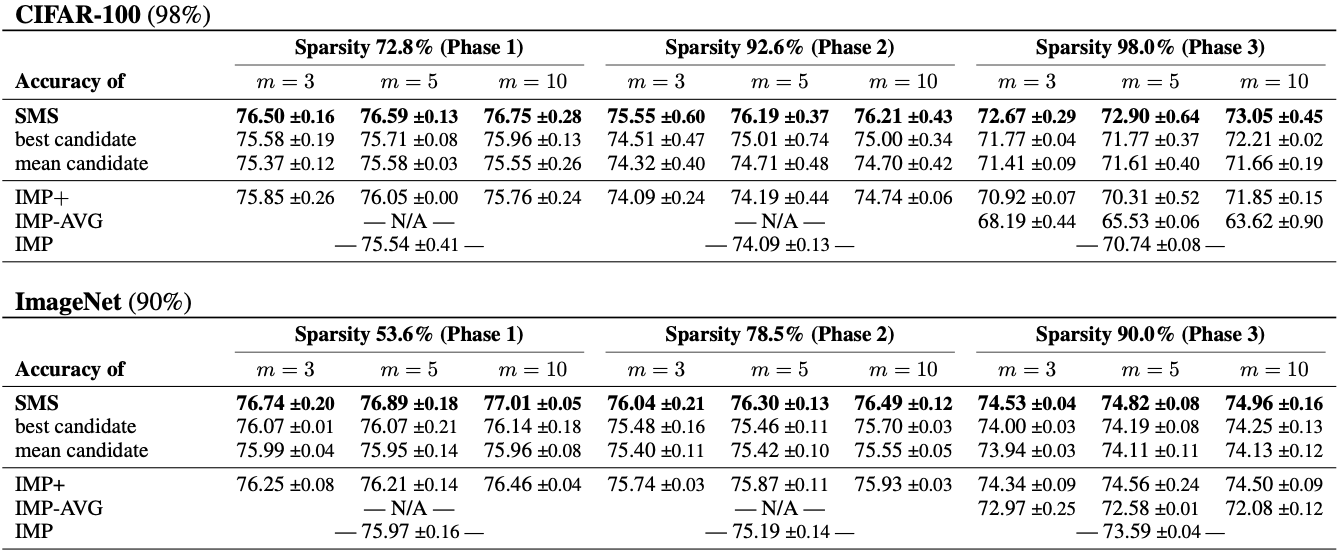Table 1. WideResNet-20 on CIFAR-100 and ResNet-50 on ImageNet: Test accuracy comparison of SMS to several baselines for target sparsities 98% (top) and 90% (bottom) given three prune-retrain cycles. Results are averaged over multiple seeds with standard deviation included. The best value is highlighted in bold.

### Conclusion

Efficient, high-performing sparse networks are crucial in resource-constrained environments. However, sparse models cannot easily leverage the benefits of parameter averaging. We addressed this issue proposing SMS, a technique that merges models while preserving sparsity, substantially enhancing IMP and outperforming multiple baselines. Please feel free to check out our paper on arXiv, where we further improve pruning during training methods such as BIMP [ZSP] and DPF [LIN] by integrating SMS. Our code is publicly available on GitHub.

### References

[HPTD] Song H., Jeff P., John T., Dally W.. Learning both weights and connections for efficient Neural Networks. In C. Cortes, N. Lawrence, D. Lee, M. Sugiyama, and R. Garnett (eds.), Advances in Neural Information Processing Systems, volume 28. Curran Associates, Inc., 2015. pdf

[LIN] Lin, T., Stich, S. U., Barba, L., Dmitriev, D., & Jaggi, M. (2020). Dynamic model pruning with feedback. arXiv preprint arXiv:2006.07253. pdf

[WM] Wortsman, Mitchell, et al. “Model soups: averaging weights of multiple fine-tuned models improves accuracy without increasing inference time.” International Conference on Machine Learning. PMLR, 2022. pdf

[YIN] Yin, L., Menkovski, V., Fang, M., Huang, T., Pei, Y., & Pechenizkiy, M. (2022, August). Superposing many tickets into one: A performance booster for sparse neural network training. In Uncertainty in Artificial Intelligence (pp. 2267-2277). PMLR. pdf

[ZSP] Zimmer, M., Spiegel, C., & Pokutta, S. (2022, September). How I Learned to Stop Worrying and Love Retraining. In The Eleventh International Conference on Learning Representations. pdf

]]>
Max Zimmer
How I Learned to Stop Worrying and Love Retraining2023-07-21T01:00:00+02:002023-07-21T01:00:00+02:00http://www.pokutta.com/blog/research/2023/07/21/abstract-BIMPTL;DR: This is an informal summary of our recent ICLR2023 paper How I Learned to Stop Worrying and Love Retraining by Max Zimmer, Christoph Spiegel, and Sebastian Pokutta, in which we reassess Iterative Magnitude Pruning (IMP) [HPTD]. Recent works [RFC, LH] demonstrate how the learning rate schedule during retraining crucially influences recovery from pruning-induced model degradation. We extend these findings, proposing a linear learning rate schedule with an adaptively chosen initial value, which we find to significantly improve post-retraining performance. We also challenge commonly held beliefs of IMP’s performance and efficiency by introducing BIMP, a budgeted IMP-variant. Our study not only enhances understanding of the retraining phase, but it also questions the prevailing belief that we should strive to avoid the need for retraining.

Written by Max Zimmer.

### Introduction and motivation

Modern Neural Networks are often highly over-parameterized, leading to significant memory demands and lengthy, computation-heavy training and inference processes. An effective solution to this is pruning, where (groups of) weights are zeroed out, significantly compressing the architecture at hand. The resulting sparse models require only a fraction of the storage and FLOPs but still perform comparably to their dense counterparts. However, as most compression strategies, pruning also comes with a tradeoff: a very heavily pruned model will normally be less performant than its dense counterpart.

Among the various strategies on when and which weights to prune, Iterative Magnitude Pruning (IMP) stands out due to its simplicity. Following the prune after training paradigm, IMP operates on a pretrained model: it starts from a well-converged model (or trains one from scratch) and then completes prune-retrain cycles iteratively or in a One Shot fashion. Pruning, eliminating a fraction of the smallest magnitude weights, usually reduces the network’s performance which has then to be recovered in a subsequent retraining phase. Typically, one performs as many cycles as are required to reach a desired degree of compression with each cycle consisting of pruning followed by retraining. IMP falls under the umbrella of pruning-instable algorithms: significant performance degradation occurs during pruning, necessitating a subsequent recovery phase through retraining. On the other hand, there exist pruning-stable algorithms. Such methods use techniques like regularization to strongly bias the regular training process, thereby driving convergence towards an almost sparse model. Consequently, the final ‘hard’ pruning step results in a minimal accuracy decline, effectively eliminating the need for retraining.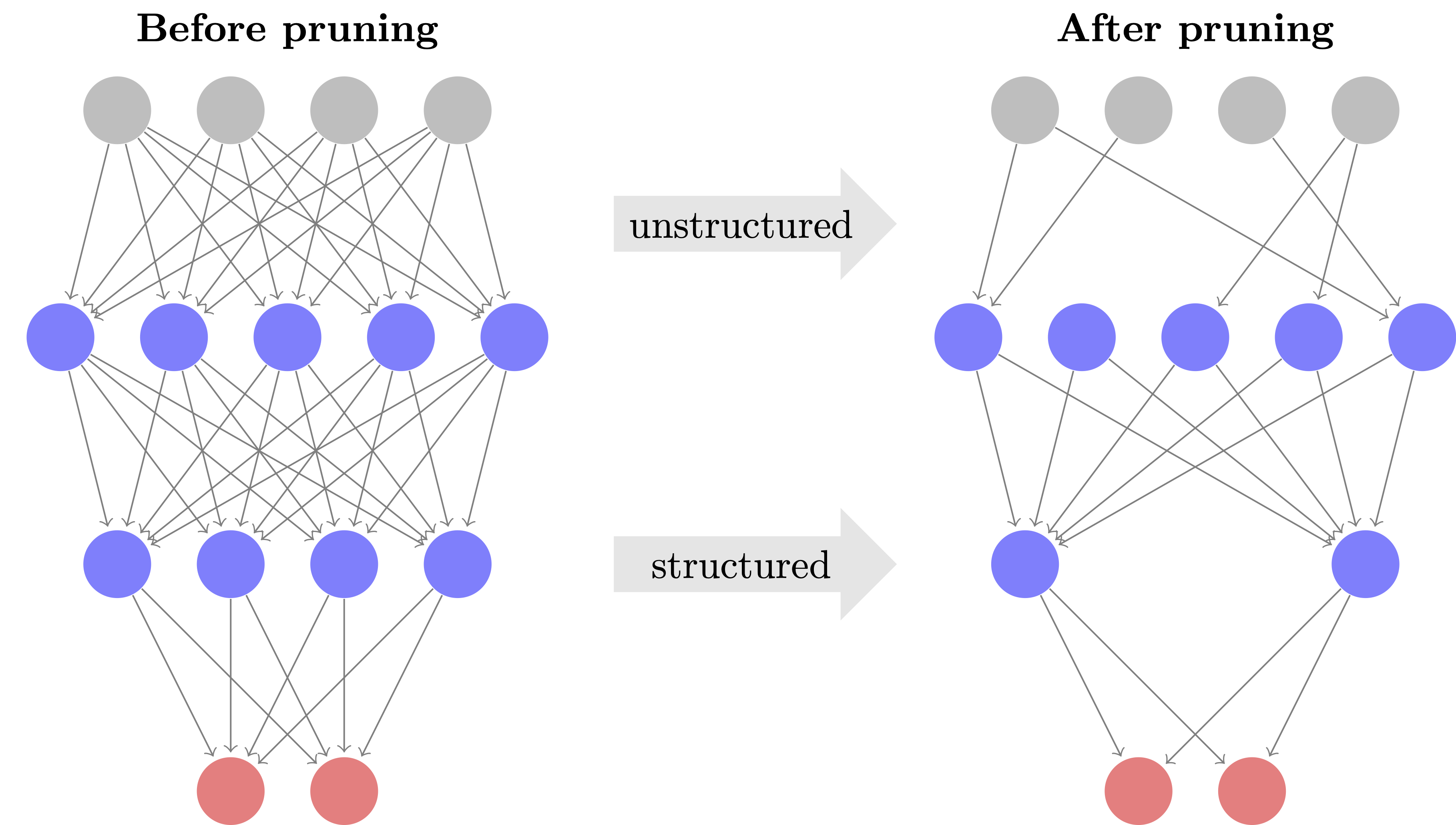Figure 1. Pruning either removes individual weights or entire groups such as neurons.

Despite its simplicity, being straightforward to implement, modularly adjustable and requiring little computational overhead per iteration, IMP is often claimed to be inferior to pruning-stable approaches, mainly for two reasons:

1. It is said to be computationally inefficient since it requires many prune-retrain cycles. Pruning-stable approaches find a sparse solution throughout regular training, while IMP first requires training an entire dense model, followed by many cycles.
2. It is said to achieve sub-optimal sparsity-accuracy-tradeoffs since it employs ‘hard’ pruning instead of ‘learning’ the sparsity pattern throughout training.

In our work, we challenge these beliefs. We begin by analyzing how to obtain empirically optimal performance in the retraining phase by leveraging an appropriate learning rate schedule.

### Improving the retraining phase

To perform retraining, we need to choose a learning rate schedule. To that end, let $$(\eta_t)_{t\leq T}$$ be the learning rate schedule of original training for $$T$$ epochs and let $$T_{rt}$$ be the number of retraining epochs, where we assume that $$\max_{t \leq T}\eta_t = \eta_1$$ and the learning rate is decaying over time, as is typically the case. Previous works have proposed several different retraining schedules:

• Finetuning (FT, [HPTD]): Use the last learning rate $$\eta_T$$ for all retraining epochs.
• Learning Rate Rewinding (LRW, [RFC]): Rewind the learning rate to epoch $$T-T_{rt}$$.
• Scaled Learning Rate Restarting (SLR, [LH]): Proportionally compress the original schedule to fit the $$T_{rt}$$ training epochs.
• Cyclic Learning Rate Restarting (CLR, [LH]): Use a 1-cycle cosine decay schedule starting from $$\eta_1$$.

While gradually improving upon their respective predecessor, we believe that these specific heuristics lack comprehensive context for their individual contributions to enhancing the retraining phase. We think that first and foremost, these findings should be interpreted in the context of Budgeted Training by Li et al. [LYR], who empirically determine optimal learning rate schedules given training for a fixed number of iterations and not until convergence as is commonly assumed. Remarkably, their findings closely resemble the development and improvement of FT, LRW, SLR and CLR. Further, Li et al. find that a linear schedule works best.

With that in mind, we hypothesize and demonstrate that these findings transfer to the retraining phase after pruning, proposing to leverage a linearly decaying schedule ($$\eta_1 \rightarrow 0$$), which we call Linear Learning Rate Restarting (LLR). Figure 1 illustrates the different schedules on a toy example.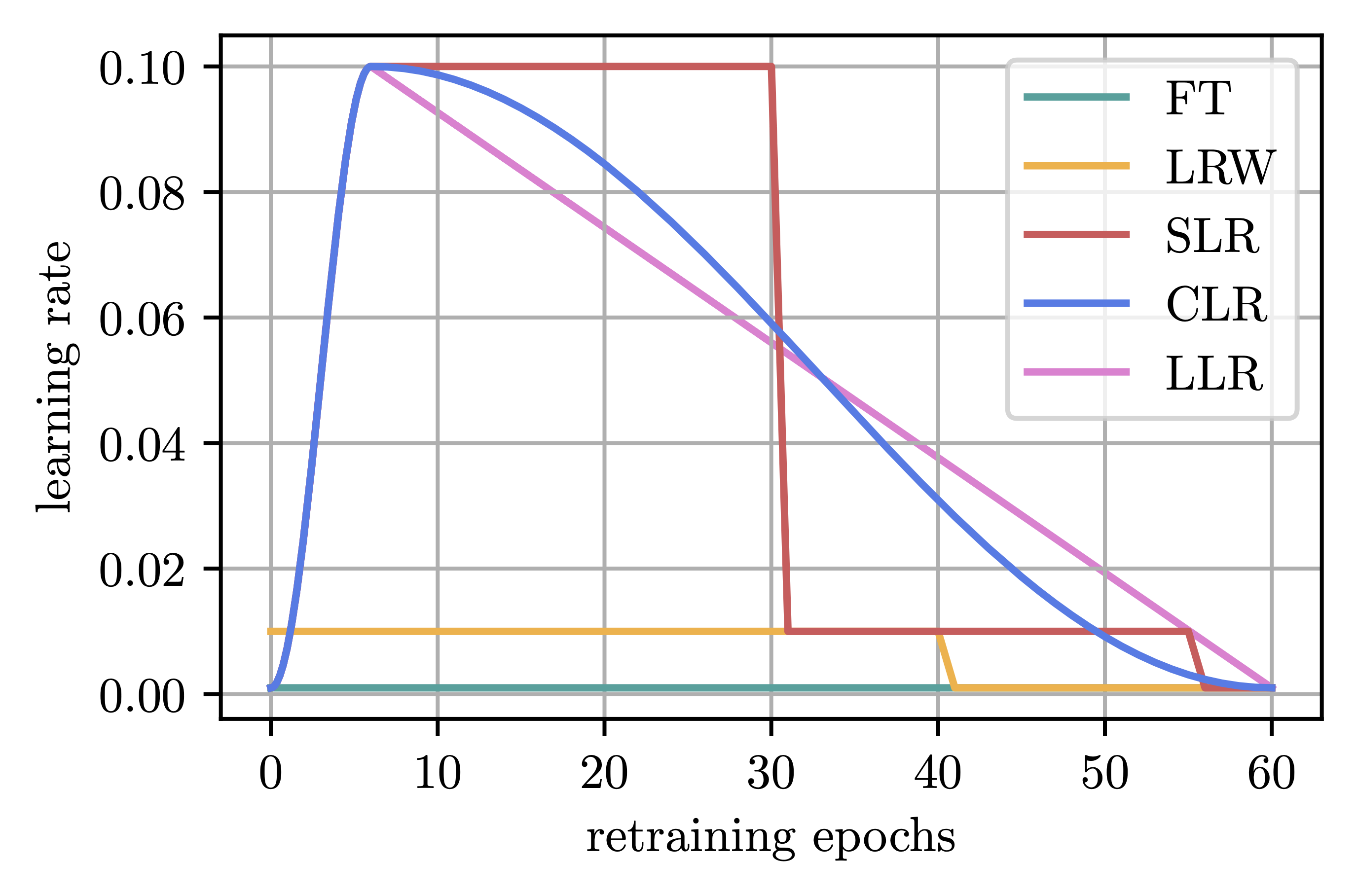Figure 2. Different retraining schedules, assuming a stepped schedule during pretraining.

Typically, the number of retraining epochs is much smaller than the length of original training, i.e., $$T_{rt} \ll T$$, and it is thus unclear whether re-increasing the learning rate to the largest value $$\eta_1$$ is desirable, given a potentially too short amount of time to recover such an aggressive restarting of the learning rate. LRW implicitly deals with this problem by coupling the magnitude of the initial learning rate to the retraining length $$T_{rt}$$. Furthermore, pruning either 20% or 90% differently disrupts the model, leading to different degrees of loss increase. We hence hypothesize that the learning rate should also reflect the magnitude of pruning impact. With larger pruning-induced losses, quicker recovery may be achieved by taking bigger steps towards the optimum. Conversely, for minor performance degradations, taking too large steps could potentially overshoot a nearby optimum.

Addressing these issues, we propose Adaptive Linear Learning Rate Restarting (ALLR), which leverages the empirically-optimal linear schedule but adaptively discounts the initial value of the learning rate by a factor $$d \in [0,1]$$ to account for both the available retraining time and the performance drop induced by pruning, instead of relying on $$\eta_1$$ as a one-fits-all solution. ALLR achieves this goal by first measuring the relative $$L_2$$-norm change in the weights due to pruning, that is after pruning an $$s \in \left( 0, 1 \right]$$ fraction of the remaining weights, we compute the normalized distance between the weight vector $$\theta$$ and its pruned version $$\theta^p$$ in the form of

\begin{equation} d_1 = \frac{\Vert \theta - \theta^p \Vert_2}{\Vert \theta \Vert_2\cdot \sqrt{s}} \in [0,1], \end{equation}

where normalization by $$\sqrt{s}$$ ensures that $$d_1$$ can actually attain the full range of values in $$[0,1]$$. We then determine $$d_2 = T_{rt} / T$$ to account for the length of the retrain phase and choose $$d \cdot \eta_1$$ as the initial learning rate for ALLR where $$d = \max (d_1, d_2)$$.

The table below shows part of the results of the effectiveness of ALLR given ResNet-50 trained on ImageNet. ALLR is able to outperform previous approaches, often by a large margin and across a wide variety of retraining budgets and target sparsities. Please see the full paper for extensive results on image classification, semantic segmentation and neural machine translation tasks and architectures, furthermore including results for structured pruning.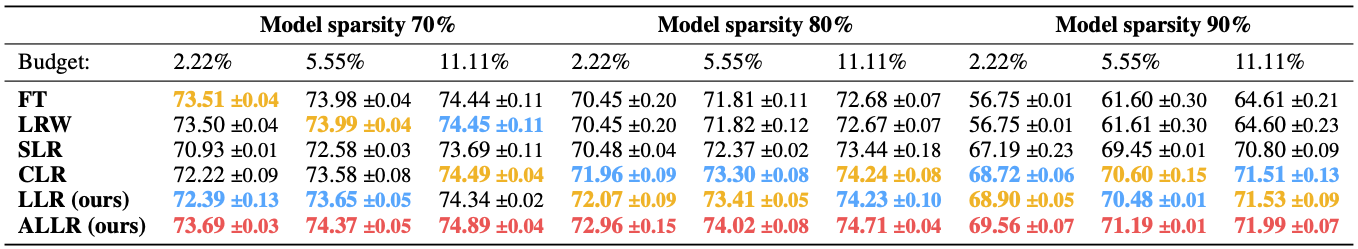Table 1. ResNet-50 on ImageNet: Performance of the different learning rate translation schemes for One Shot IMP for target sparsities of 70%, 80% and 90% and retrain times of 2.22% (2 epochs), 5.55% (5 epochs) and 11.11% (10 epochs) of the initial training budget. Results are averaged over two seeds with the standard deviation indicated. The first, second, and third best values are highlighted.

### Challenging commonly held beliefs about IMP

Having established that we can recover pruning-induced losses more effectively by taking proper care of the learning rate, we return to the two drawbacks of IMP when compared to pruning-stable methods, which as opposed to IMP are able to produce a sparse model starting from random initialization without needing further retraining. To verify whether the claimed disadvantages of IMP are backed by evidence, we propose Budgeted IMP (BIMP), where the same lessons we previously derived from Budgeted Training for the retraining phase of IMP are applied to the initial training of the network. Given a budget of $$T$$ epochs, BIMP simply trains a network for some $$T_0 < T$$ epochs using a linear schedule and then applies IMP with ALLR on the output for the remaining $$T-T_0$$ epochs. BIMP obtains a pruned model from scratch within the same budget as pruning-stable methods, while still maintaining the key characteristics of IMP, i.e.,

• we prune ‘hard’ and do not allow weights to recover in subsequent steps, and
• we do not impose any particular additional implicit bias during either training or retraining.

The following table compares BIMP to a variety of pruning-stable methods trained on CIFAR-10 (above) and ImageNet (below), given three different levels of sparsity. Despite inheriting the properties of IMP, we find that BIMP reaches results on-par to or better than much more complex methods. Further, our experiments show that BIMP is among the most efficient approaches as measured by the number of images processed per training iteration (second column).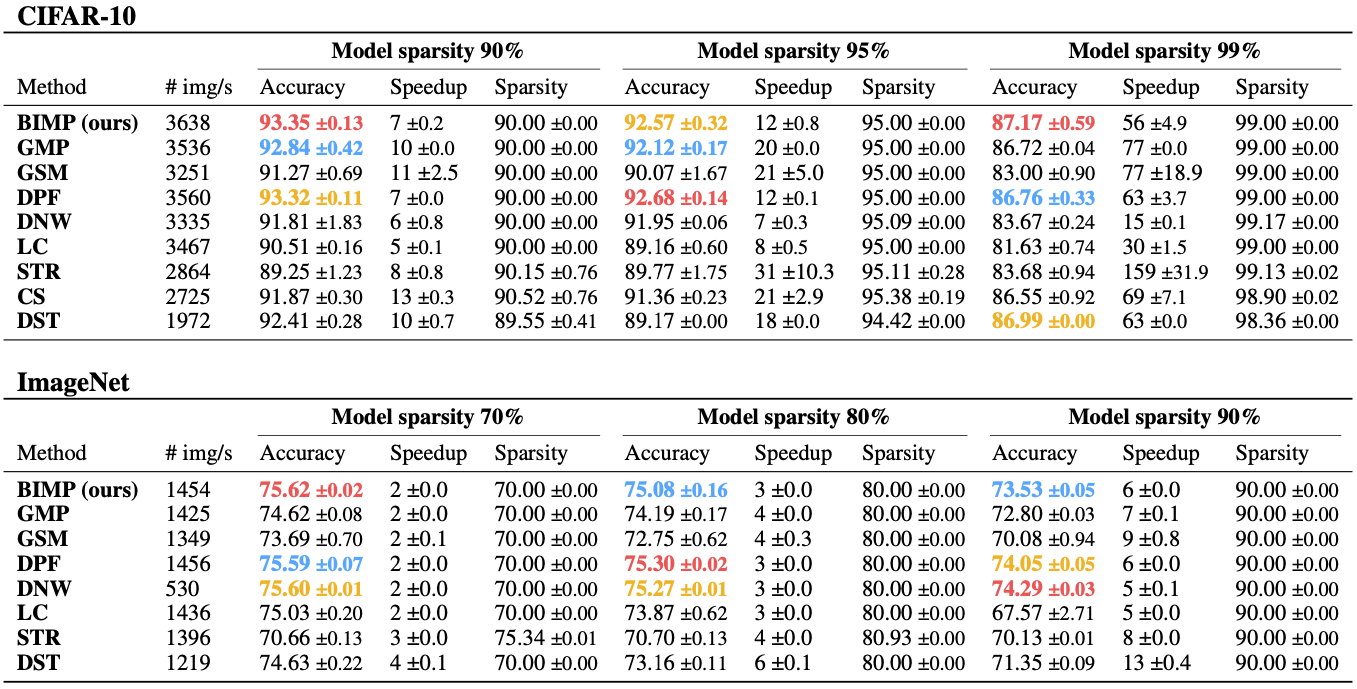Table 2. ResNet-56 on CIFAR-10 (above) and ResNet-50 on ImageNet (below): Comparison between BIMP and pruning-stable methods when training for goal sparsity levels of 90%, 95%, 99% (CIFAR-10) and 70%, 80%, 90% (ImageNet), denoted in the main columns. Each subcolumn denotes the Top-1 accuracy, the theoretical speedup and the actual sparsity achieved by the method. Further, we denote the images-per-second throughput during training, i.e., a higher number indicates a faster method. All results are averaged over multiple seeds and include standard deviations. The first, second, and third best values are highlighted.

### Conclusion

Contrary to the prevailing notion of IMP’s inferiority, our work reveals that when the learning rate is appropriately managed, this arguably simplest sparsification approach can outperform much more complex methods in terms of both performance and efficiency. Further, we put the development and benefits of different retraining schedules into perspective and provide a strong alternative with ALLR. However, let us emphasize that the goal of our work was not to suggest yet another acronym and claim it to be the be-all and end-all of network pruning, but rather to emphasize that IMP can serve as a strong, easily implemented, and modular baseline, which should be considered before suggesting further convoluted novel methods.

You can find the paper on arXiv. Our code is publicly available on GitHub. Please also check out our ICLR2023 poster and SlidesLive presentation for more information.

### References

[HPTD] Song Han, Jeff Pool, John Tran, and William Dally. Learning both weights and connections for efficient Neural Networks. In C. Cortes, N. Lawrence, D. Lee, M. Sugiyama, and R. Garnett (eds.), Advances in Neural Information Processing Systems, volume 28. Curran Associates, Inc., 2015. pdf

[LH] Duong Hoang Le and Binh-Son Hua. Network pruning that matters: A case study on retraining variants. In International Conference on Learning Representations, 2021. pdf

[LYR] Mengtian Li, Ersin Yumer, and Deva Ramanan. Budgeted training: Rethinking deep neural network training under resource constraints. In International Conference on Learning Representations, 2020. pdf

[RFC] Alex Renda, Jonathan Frankle, and Michael Carbin. Comparing rewinding and fine-tuning in neural network pruning. In International Conference on Learning Representations, 2020. pdf

]]>
Max Zimmer
Alternating Linear Minimization2023-07-04T06:00:00+02:002023-07-04T06:00:00+02:00http://www.pokutta.com/blog/research/2023/07/04/abstract-alternating-minimizationsTL;DR: This is an informal overview of our recent paper Alternating Linear Minimization: Revisiting von Neumann’s alternating projections by Gábor Braun, Sebastian Pokutta, and Robert Weismantel where we replace the projections in von Neumann’s alternating projections algorithm with linear minimizations, which are much cheaper, while maintaining essentially the same convergence rate.

## Why you might care

In 1933, von Neumann established a fundamental algorithm to compute (the approximation of) a point within the intersection of two convex sets using an approach known as alternating projections [vN49]. This process involves sequential projections onto one set followed by the other. The feasibility of execution of this algorithm, however, is contingent upon the availability of (computationally efficient) projection operators for both convex sets.

In our paper [BPW22], our objective is to examine a scenario with more limited resources, specifically where access is restricted to linear minimization oracles over the convex sets. Linear minimization is often much cheaper than projection, in particular when we are a concerned with complicated constraints. We present a new algorithm tailored to these assumptions. Despite using the much simpler linear minimization oracles, our algorithm (approximately) identifies a point within the intersection of the two convex sets, while essentially maintaining the same convergence rate, generalizing von Neumann’s original result. Moreover, the algorithm can be made exact (i.e., exactly decide if $P \cap Q \neq \emptyset$) in the case of e.g., polytopes.

## von Neumann’s algorithm and convergence

Let us briefly recall von Neumann’s original algorithm. It is really straightforward, alternatingly projecting on the respective sets:

von Neumann’s Alternating Projections (POCS).
Requirements: Point $$y_0 \in \RR^n$$, $$\Pi_P$$ projector onto $$P \subseteq \RR^n$$ and $$\Pi_Q$$ projector onto $$Q \subseteq \RR^n$$.
Output: Iterates $$x_1, y_1, \dots$$
for $$t = 0, 1, 2, \dots$$
$$\quad x_{t+1} = Π_P(y_t)$$
$$\quad y_{t+1} = Π_Q(x_{t+1})$$
end for

Showing convergence for this algorithm follows a simple but powerful euclidean norm expansion argument; we reproduce the proof here for completeness. Suppose that $$u \in P \cap Q \neq \emptyset$$. Then:

\begin{align*} \norm{y_t - u}^2 & = \norm{y_t - x_{t+1} + x_{t+1} - u}^2 = \norm{y_t - x_{t+1}}^2 + \norm{x_{t+1} - u}^2 - 2 \underbrace{\langle x_{t+1} - y_t, x_{t+1} - u \rangle}_{\leq 0} \\ & \geq \norm{y_t - x_{t+1}}^2 + \norm{x_{t+1} - u}^2 = \norm{y_t - x_{t+1}}^2 + \norm{x_{t+1} - y_{t+1} + y_{t+1} - u}^2 \\ & = \norm{y_t - x_{t+1}}^2 + \norm{x_{t+1} - y_{t+1}}^2 + \norm{y_{t+1} - u}^2 - 2 \underbrace{\langle y_{t+1} - x_{t+1}, y_{t+1} - u \rangle}_{\leq 0} \\ & \geq \norm{y_t - x_{t+1}}^2 + \norm{x_{t+1} - y_{t+1}}^2 + \norm{y_{t+1} - u}^2, \end{align*}

where the non-positivity of the scalar products follows from the fact that these are precisely the first-order optimality conditions of the projection operation. The above can be rearranged to

$\norm{y_t - u}^2 - \norm{y_{t+1} - u}^2 \geq \norm{y_t - x_{t+1}}^2 + \norm{x_{t+1} - y_{t+1}}^2.$

Now we are essentially done: Starting from

$\norm{y_t - u}^2 - \norm{y_{t+1} - u}^2 \geq \norm{y_t - x_{t+1}}^2 + \norm{x_{t+1} - y_{t+1}}^2.$

1) We simply sum up:

$\sum_{t = 0}^{T-1} \left(\norm{y_t - u}^2 - \norm{y_{t+1} - u}^2\right) \geq \sum_{t = 0}^{T-1} \left( \norm{y_t - x_{t+1}}^2 + \norm{x_{t+1} - y_{t+1}}^2 \right).$

2) This implies, through telescoping:

$\norm{y_0 - u}^2 \geq \sum_{t = 0}^{T-1} \left( \norm{y_t - x_{t+1}}^2 + \norm{x_{t+1} - y_{t+1}}^2\right).$

3) We divide by $T$, obtaining:

$\frac{\norm{y_0 - u}^2}{T} \geq \frac{1}{T} \sum_{t = 0}^{T-1} \left( \norm{y_t - x_{t+1}}^2 + \norm{x_{t+1} - y_{t+1}}^2 \right) \geq \norm{x_{T} - y_{T}}^2,$

as distances are non-increasing. This completes the convergence proof and (after a little more work) we obtain:

Proposition (von Neumann, 1949 + minor perturbations).
Let $$P$$ and $$Q$$ be compact convex sets with $$P \cap Q \neq \emptyset$$ and let $$x_1, y_1, \dots, x_T, y_T \in \RR^n$$ be the sequence of iterates of von Neumann’s algorithm. Then the iterates converge: $$x_{t} \to x$$ and $$y_{t} \to y$$ to some $$x \in P$$ and $$y \in Q$$. Furthermore, we have: $\norm{x_{T} - y_{T}}^{2} \leq \frac{1}{T} \sum_{t = 0}^{T-1} \left(\norm{y_{t} - x_{t+1}}^{2} + \norm{x_{t+1} - y_{t+1}}^{2} \right) \leq \frac{\operatorname{dist}(y_0, P \cap Q)^{2}}{T}.$

## Alternating Linear Minimizations

Now suppose we can access the feasible regions $P$ and $Q$ only by means of linear minimization oracles, i.e., we can compute only $$x \leftarrow \arg\min_{u \in P} \langle c, u \rangle$$ and $$y \leftarrow \arg\min_{w \in Q} \langle c, w \rangle$$, for any given $c \in \RR^n$. Then it turns out we can formulate an algorithm very close to von Neumann’s original algorithm, but only relying on linear minimizations as access to $P$ and $Q$:

Alternating Linear Minimizations (ALM).
Requirements: Points $$x_{0} \in P$$, $$y_{0} \in Q$$, LMO over $$P, Q \subseteq \RR^{n}$$.
Output: Iterates $$x_1, y_1, \dots \in \RR^n$$.
for $$t = 0, 1, 2, \dots$$
$$\quad u_{t} = \arg\min_{x \in P} \langle x_{t} - y_{t}, x \rangle$$
$$\quad x_{t+1} = x_{t} + \frac{2}{t+2} \cdot (u_{t} - x_{t})$$
$$\quad v_{t} = \arg\min_{y \in Q} \langle y_{t} - x_{t+1}, y \rangle$$
$$\quad y_{t+1} = y_{t} + \frac{2}{t+2} \cdot (v_{t} - y_{t})$$
end for

Effectively we are doing a single Frank-Wolfe step on each set and then repeat. We obtain the following convergence rates:

Proposition (Intersection of two sets).
Let $$P$$ and $$Q$$ be compact convex sets. Then ALM generates iterates $$z_t = \frac{1}{2} (x_t + y_t)$$, such that: $\max\{\operatorname{dist}(z_t, P)^2, \operatorname{dist}(z_t, Q)^2\} \leq \frac{\norm{x_{t} - y_{t}}^{2}}{4} \leq \frac{(1 + 2 \sqrt{2}) (D_{P}^{2} + D_{Q}^{2})}{t+2} + \frac{\operatorname{dist}(P,Q)^{2}}{4},$ as primal convergence guarantee and also, and $\min_{1 \leq t \leq T} \max_{x \in P, y \in Q} \norm{x_{t} - y_{t}}^{2} - \langle x_{t} - y_{t}, x - y \rangle \leq \frac{6.75 (1 + 2 \sqrt{2})}{T + 2} (D_{P}^{2} + D_{Q}^{2}),$ as dual convergence guarantee.

This guarantee can also be used to certify disjointness of $P$ and $Q$. Moreover, in the case of $P$ and $Q$ being polytopes, the algorithm and guarantee can be made exact in the sense of exactly deciding whether $P \cap Q \neq \emptyset$ (rather than doing so only approximately) by combination with linear programming; see [BPW22] for details.

For completeness let us briefly compare the obtained rates to those of von Neumann’s original algorithm:

Remark (Comparison to von Neumann’s alternating projection algorithm).
For simplicity, consider the case where $$P \cap Q \neq \emptyset$$.
After minor reformulation, von Neumann’s alternating projection method yields: $\min_{t=0, \dots, T-1} \max{\operatorname{dist}(z_{t+1}, P)^2, \operatorname{dist}(z_{t+1}, Q)^2} \leq \frac{\operatorname{dist}(y_0, P \cap Q)^{2}}{T}.$ Alternating Linear Minimization yields: $\max{\operatorname{dist}(z_T, P)^2, \operatorname{dist}(z_T, Q)^2} \leq \frac{(1 + 2 \sqrt{2}) (D_{P}^{2} + D_{Q}^{2})}{T+2}.$

As we can see, the convergence rate of ALM is essentially identical to that of von Neumann’s algorithm except for different ($P$ and $Q$ dependent) constants. The ALM algorithm also works quite well in actual computations but that is beyond the scope of that specific paper.

### References

[vN49] Von Neumann, J. (1949). On rings of operators. Reduction theory. Annals of Mathematics, 401-485. [pdf]

[BPW22] Braun, G., Pokutta, S., & Weismantel, R. (2022). Alternating Linear Minimization: Revisiting von Neumann’s alternating projections. [arxiv].

]]>
Sebastian Pokutta
Improved local models and new Bell inequalities via Frank-Wolfe algorithms2023-05-05T06:00:00+02:002023-05-05T06:00:00+02:00http://www.pokutta.com/blog/research/2023/05/05/BellPolytopesFWTL;DR: This is an informal overview of our recent paper Improved local models and new Bell inequalities via Frank-Wolfe algorithms by Sébastien Designolle, Gabriele Iommazzo, Mathieu Besançon, Sebastian Knebel, Patrick Gelß, and Sebastian Pokutta, where we use Frank-Wolfe algorithms to improve several non-locality constants.

Written by Sébastien Designolle.

Comment (Sebastian Pokutta): This research arose out of peculiar conincidence with me preparing the (still unpublished!) follow-up blog post for Quantum Computing for the Uninitiated, which was about the Bell polytope and Sébastien visiting ZIB right at that time. It is funny how these things happen sometimes.

## Frank-Wolfe in Bell polytopes

Today we’re using Frank-Wolfe in a specific polytope arising from quantum information. This should give an introductory foretaste of our recent preprint.

Consider a bipartite scenario in which Alice and Bob, as the parties are usually referred to, receive questions and give answers. By repeating this many rounds, you can construct the joint probability characterising their strategy. If this strategy only makes use of classical resources, the resulting joint probability must be in the convex hull of deterministic strategies (those mapping the questions directly to the answers). Interestingly, if Alice and Bob are allowed to exploit the correlations arising from entangled quantum systems, they can get a point outside of this polytope. This result is the essence of Bell’s theorem, from a paper published in 1964 in an obscure journal . Of course, his setup and phrasing are a bit different than the one just briefly sketched above, but the fundamental idea remains the same and led to an entire branch of research working on this so-called quantum nonlocality. This field was recently under the spotlights as the experimental demonstrations of Bell’s theorem were awarded the Nobel prize in physics in 2022.

### CHSH

But let’s give an example to be a bit more specific; as often this will be the celebrated CHSH inequality . So we fix the number of questions to be two (labelled by $$x$$ for Alice and $$y$$ for Bob, both being 1 or 2) and the answers to be $$\pm1$$. For simplicity of the presentation, we further assume that these answers are balanced (-1 and +1 are equally likely) so that the only things that count are the expectation values $$\langle A_xB_y\rangle$$ of the product of Alice’s and Bob’s answers. Then the polytope we were mentioning before, called the local polytope and denoted $$\mathcal{L}$$, has eight vertices:

$\begin{pmatrix} - & -\\ - & - \end{pmatrix}\quad \begin{pmatrix} + & +\\ + & + \end{pmatrix}\quad \begin{pmatrix} - & +\\ - & + \end{pmatrix}\quad \begin{pmatrix} + & -\\ + & - \end{pmatrix}\quad \begin{pmatrix} - & -\\ + & + \end{pmatrix}\quad \begin{pmatrix} + & +\\ - & - \end{pmatrix}\quad \begin{pmatrix} - & +\\ + & - \end{pmatrix}\quad \begin{pmatrix} + & -\\ - & + \end{pmatrix},$

where the lines of these correlation matrices are labelled by $$x$$ and the columns by $$y$$. These deterministic strategies are denoted $$\mathbf{d}$$ in the following.

With a quantum strategy whose detail does not really matter here, the following correlation matrix can be obtained:

$\mathbf{p}=\frac{1}{\sqrt2}\begin{pmatrix} + & +\\ + & - \end{pmatrix},$

which is indeed outside of the local polytope. Actually we can go a bit further than this dichotomic criterion inside/outside and ask how far away from the polytope this point is, in the following sense: by moving along the line between the point and the center of the polytope (the zero matrix), there is a threshold at which we enter $$\mathcal{L}$$. In the CHSH example, the corresponding parameter is $$v^\ast=1/\sqrt2$$. To show this, we need two ingredients:

a) a separating hyperplane, called a Bell inequality, showing that any $$v > v^\ast$$ leads to a point outside $$\mathcal{L}$$:

$\mathrm{tr}\left[\begin{pmatrix} + & +\\ + & - \end{pmatrix}\mathbf{d}\right]\leqslant2 \quad\text{while}\quad \mathrm{tr}\left[\begin{pmatrix} + & +\\ + & - \end{pmatrix}\mathbf{p}\right]=2\sqrt2.$

b) a convex decomposition of $$v^\ast \mathbf{p}$$, called a local model, that explicitly proves that $$v^\ast\mathbf{p} \in\mathcal{L}$$:

$\frac{1}{\sqrt2}\mathbf{p}=\frac12\begin{pmatrix} + & +\\ + & - \end{pmatrix} =\frac14\left[ \begin{pmatrix} + & +\\ + & + \end{pmatrix}+ \begin{pmatrix} + & -\\ + & - \end{pmatrix}+ \begin{pmatrix} + & +\\ - & - \end{pmatrix}+ \begin{pmatrix} - & +\\ + & - \end{pmatrix} \right].$

### Nonlocality threshold

From a physical perspective, this quantity $$v^\ast$$ also has a meaning: it corresponds to the robustness of the quantum strategy to white noise on the shared quantum state. And, even if the actual experiment naturally gets way more difficult to implement as the number $$m$$ of measurements increases, the question of finding Bell inequalities with high robustnesses (that is, low $$v^\ast$$) is of important theoretical relevance. So our main problem is the following: find the noise threshold at which our two-qubit state (a so-called singlet state whose form is irrelevant for the discussion here) cannot be used to observe nonlocality, no matter how many (projective) measurements we use.

At this game, the CHSH inequality described above, published in 1969, is a good contender. Actually, the question of finding a better inequality remained open until 2008, where an inequality with $$v^\ast<1/\sqrt2$$ was found, with 465 possible measurements! With this example, you can probably foresee why our general question is so hard: the number $$m$$ of measurements is not fixed, and the optimal robustness can only happen in the limit of an infinite number of them. And the structure of the local polytope gets complex very quickly with $$m$$: it has $$2^{2m-1}$$ vertices in a space of dimension $$m^2$$, so that even enumerating all vertices is very quickly impossible. Note that a complete list of its facets is only known up to $$m=4$$.

### Frank-Wolfe algorithms

This is where Frank-Wolfe (FW) algorithms come handy, in that it gives a way to construct:

• a sparse decomposition of a point inside $$\mathcal{L}$$,
• a separating hyperplane for a point outside $$\mathcal{L}$$.

The idea is to solve, for an initial visibility $$v_0$$, the following minimisation problem:

$\min_{\mathbf{x}\in\mathcal{L}}\frac{1}{2}\|\mathbf{x}-v_0\mathbf{p}\|_2^2,$

which converges to $$\mathbf{x}$$ if $$\mathbf{x} \in \mathcal{L}$$ and to its orthogonal projection onto $$\mathcal{L}$$ otherwise.

This approach in itself is not particularly new as it was already used in 2016 for this specific problem. What is new, however, is to use an efficient algorithm to drastically accelerate the convergence of previous works relying of vanilla FW (and thus suffering from zigzagging). In our case, we implemented the blended pairwise conditional gradient algorithm . This speeds up things immensely, as the costly Linear Minimisation Oracle (LMO) is not called at each step but only when the progress achievable by means of previously computed atoms becomes too low.

### Linear Minimisation Oracle

In our case, the LMO looks like

$\mathrm{max}_{\vec{a},\vec{b} \in \{\pm1\}^m} \sum_{x,y \in [m]} a_x M_{xy} b_y,$

where $$\{M_{xy}\}_{xy}$$ is the direction along which we want to minimise in the local polytope. From a physical perspective, this “simply” amounts to finding the local bound of a Bell inequality encoded by the coefficients $$M_{xy}$$. However, this problem is NP-hard and going beyong $$m\approx100$$ is extremely challenging.

Fortunately though, a good enough heuristic is all we need in general. It turns out that starting from a random choice of $$\vec{b}$$ and alternatately minimising over $$\vec{a}$$ and $$\vec{b}$$ gives rather good solutions, at least when doing this process many times (a few thousands in practice). There is absolutely no guarantee of optimality, but as soon as FW can use this heuristic LMO to make progress, this approach is sufficient in the case $$\mathbf{x} \in \mathcal{L}$$. Why not when $$\mathbf{x} \not\in \mathcal{L}$$? Because it is necessary to solve at least one instance to optimality in order to obtain a valid separating hyperplane.

For this last problem, we also came with a solution to go a bit beyond existing methods: by reformulating the LMO as a Quadratic Unconstrained Binary Optimisation (QUBO) instance, we could use a recently developed solver , which is unfortunately closed source, to solve to optimality an instance with $$m=97$$ measurements.

### Results

With the overview of our methodology done above we can state the results obtained in the bipartite case, that is, with correlation matrices as presented above. You’re most likely not familiar with the problem so it’s worth giving some historical context on the progress made on both lower and upper bounds over the years.

$$v_c^\mathrm{Wer}$$ $$m$$ Year
0.7071 2 1969
0.7056 465 2008
0.7054 $$\infty$$ 2015
0.7012 42 2016
0.6964 90 2017
0.6961 97 Our
0.6875 $$406\sim\infty$$ work
0.6829 $$625\sim\infty$$ 2017
0.6595 $$\infty$$ 2006
0.5 $$\infty$$ 1989

A few comments about the lower bounds are in order: they contain an extra step (not explained here) that goes from a finite number of measurements to all projective measurements. The idea is that we can simulate the former by means of the latter, up to some factor that gets closer to 1 as the number of measurements increases. Part of the improvements that we brought to the problem came from the choice of these measurements; this explains, by the way, why we use less measurements than the previous lower bound while still beating it. Without going into details here, we used symmetric polyhedra in the Bloch sphere; they can be easily constructed and visualised here (try, for instance, the recipe Au3I which gives an idea of the kind of structures that we used).

We don’t present the multipartite results here as most of you don’t care, but the entire approach naturally generalises to correlation tensors of arbitrary order (meaning any number of parties). And the known results in this direction were quite scarce, so that our bounds provide way more insight than the tightening (arguably minute to some extent) presented in the table above. For instance, we give the first proof that, for three-qubit states, the W state is less nonlocal than the GHZ state.

### References

 John Bell. On the Einstein-Podolsky-Rosen paradox. Physics Physique Fizika 1, 195-200 (1964).

 Clauser, Horne, Shimony, Holt. Proposed experiment to test local hidden-variable theories. Phys. Rev. Lett. 23, 880 (1969).

 Tsuji, Tanaka, Pokutta. Sparser kernel herding with pairwise conditional gradients without swap steps. arXiv:2110.12650 (2021).

 Rehfeldt, Koch, Shinano. Faster exact solution of sparse MaxCut and QUBO problems. arXiv:2202.02305 (2022).

]]>
Sébastien Designolle
Sh**t you can do with the euclidean norm2022-12-29T05:00:00+01:002022-12-29T05:00:00+01:00http://www.pokutta.com/blog/research/2022/12/29/shXXt-you-can-do-2normTL;DR: Some of my favorite arguments all following from a simple expansion of the euclidean norm and averaging.

This post is about a particular argument involving the euclidean norm. The basic idea is always the same: we expand the euclidean norm akin to the binomial formula and then do some form of averaging. We really focus on this specific argument alone here: It is not guaranteed that the estimations are optimal (although they often are) and also sometimes the argument can be generalized, replacing the euclidean norm, e.g., with the respective Bregmann divergences.

## (Sub-)Gradient Descent and Online Learning

Our first example is (sub-)gradient descent without any fluff; see Cheat Sheet: Subgradient Descent, Mirror Descent, and Online Learning for a more detailed discussion and its pecularities. Consider the basic iterative scheme:

$\tag{subGD} x_{t+1} \leftarrow x_t - \eta \partial f(x_t),$

where $f$ is a not necessarily smooth but convex function and $\partial f$ are its (sub-)gradients. We show how we can establish convergence of the above scheme to an (approximately) optimal solution $x_T$ to $\min_{x \in \RR^n} f(x)$ with $x^\esx$ being an optimal solution to $\min_{x \in \RR^n} f(x)$. To this end, we will first expand the euclidean norm as follows; basically the binomial formula:

\begin{align*} \norm{x_{t+1} - x^\esx}^2 & = \norm{x_t - \eta \partial f(x_t) - x^\esx}^2 \\ & = \norm{x_t - x^\esx}^2 - 2 \eta \langle \partial f(x_t), x_t - x^\esx\rangle + \eta^2 \norm{\partial f(x_t)}^2. \end{align*}

This can be rearranged to

\begin{align*} \tag{subGD-iteration} 2 \eta \langle \partial f(x_t), x_t - x^\esx\rangle & = \norm{x_t - x^\esx}^2 - \norm{x_{t+1} - x^\esx}^2 + \eta^2 \norm{\partial f(x_t)}^2. \end{align*}

Whenever we have an expression of the form (subGD-iteration), we can typically complete the convergence argument in three steps. We first 1) add up those expressions for $t = 0, \dots, T-1$ and 2) telescope to obtain:

\begin{align*} \sum_{t = 0}^{T-1} 2\eta \langle \partial f(x_t), x_t - x^\esx\rangle & = \norm{x_0 - x^\esx}^2 - \norm{x_{T} - x^\esx}^2 + \sum_{t = 0}^{T-1} \eta^2 \norm{\partial f(x_t)}^2 \\ & \leq \norm{x_0 - x^\esx}^2 + \sum_{t = 0}^{T-1} \eta^2 \norm{\partial f(x_t)}^2. \end{align*}

For simplicity, let us further assume that $\norm{\partial f(x_t)} \leq G$ for all $t = 0, \dots, T-1$ for some $G \in \RR$. Then the above simplifies to:

\begin{align*} 2\eta \sum_{t = 0}^{T-1} \langle \partial f(x_t), x_t - x^\esx\rangle & \leq \norm{x_0 - x^\esx}^2 + \eta^2 T G^2 \\ \Leftrightarrow \sum_{t = 0}^{T-1} \langle \partial f(x_t), x_t - x^\esx\rangle & \leq \frac{\norm{x_0 - x^\esx}^2}{2\eta} + \frac{\eta}{2} T G^2. \end{align*}

At this point we could minimize the right-hand side by setting

$\eta \doteq \frac{\norm{x_0 - x^\esx}}{G} \sqrt{\frac{1}{T}},$

\begin{align*} \sum_{t = 0}^{T-1} \langle \partial f(x_t), x_t - x^\esx\rangle & \leq G \norm{x_0 - x^\esx} \sqrt{T}, \end{align*}

however for this we would need to know $G$ and $\norm{x_0 - x^\esx}$, which is often not practical but we can simply set $\eta \doteq \sqrt{\frac{1}{T}}$, which is good enough, and obtain:

\begin{align*} \sum_{t = 0}^{T-1} \langle \partial f(x_t), x_t - x^\esx\rangle & \leq \frac{G^2 + \norm{x_0 - x^\esx}^2}{2} \sqrt{T}, \end{align*}

i.e., not knowing the parameters leads to the arithmetic mean rather than the geomtric mean of the coefficients.

Then finally, 3) we average by dividing both sides by $T$. Together with convexity and the subgradient property it holds that $f(x_t) - f(x^\esx) \leq \langle \partial f(x_t), x_t - x^\esx\rangle$ and we can conclude:

\begin{align*} \tag{convergenceSG} f(\bar x) - f(x^\esx) & \leq \frac{1}{T} \sum_{t = 0}^{T-1} f(x_t) - f(x^\esx) \\ & \leq \frac{1}{T} \sum_{t = 0}^{T-1} \langle \partial f(x_t), x_t - x^\esx\rangle \\ & \leq \frac{G^2 + \norm{x_0 - x^\esx}^2}{2} \frac{1}{\sqrt{T}}, \end{align*}

where $\bar x \doteq \frac{1}{T} \sum_{t=0}^{T-1} x_t$ is the average of all iterates and the first inequality directly follows from convexity. As such we have effectively shown a $O(1/\sqrt{T})$ convergence rate for our subgradient descent algorithm (subGD).

It is useful to observe that the algorithm actually minimizes the average of the dual gaps at points $x_t$ given by $\langle \partial f(x_t), x_t - x^\esx\rangle$ and since the average of the dual gaps upper bounds the primal gap of the average point (via convexity) primal convergence follows. Moreover, this type of argument also allows us to obtain guarantees for online learning algorithms by simply observing that we could have used a different function $f$ for each $t$ in (subGD-iteration); for details see Cheat Sheet: Subgradient Descent, Mirror Descent, and Online Learning.

## Von Neumann’s alternating projections

Next we come to von Neumann’s Alternating Projections algorithm that he formulated first in his lecture notes in 1933 and which was later (re-)printed in [vN] in 1949. Given two compact convex sets $P$ and $Q$ with associated projection operators $\Pi_P$ and $\Pi_Q$, our goal is to find a point $x \in P \cap Q$; originally von Neumann formulated the argument for linear spaces but it is pretty much immediate that his argument holds much more generally. His algorithm is quite straightforward basically, alternatingly projecting onto the respective sets:

von Neumann’s Alternating Projections [vN]
Input: Point $y_{0} \in \RR^n$, $\Pi_P$ projector onto $P \subseteq \RR^n$ and $\Pi_Q$ projector onto $Q \subseteq \RR^n$.
Output: Iterates $x_1,y_1 \dotsc \in \RR^n$
For $t = 1, \dots$ do:
$\quad x_{t+1} \leftarrow \Pi_P(y_{t})$
$\quad y_{t+1} \leftarrow \Pi_Q(x_{t+1})$

Now suppose $P \cap Q \neq \emptyset$ and let $u \in P \cap Q$ be arbitrary. We will show that the algorithm converges to a point in the intersection. The argument is quite similar to the above. We consider a given iterate, add $0$, and then use the binomial formula (and repeat):

\begin{align*} \norm{y_t - u}^2 & = \norm{y_t - x_{t+1} + x_{t+1} - u}^2 = \norm{y_t - x_{t+1}}^2 + \norm{x_{t+1} - u}^2 - 2 \underbrace{\langle x_{t+1} - y_t, x_{t+1} - u \rangle}_{\leq 0} \\ & \geq \norm{y_t - x_{t+1}}^2 + \norm{x_{t+1} - u}^2 = \norm{y_t - x_{t+1}}^2 + \norm{x_{t+1} - y_{t+1} + y_{t+1} - u}^2 \\ & = \norm{y_t - x_{t+1}}^2 + \norm{x_{t+1} - y_{t+1}}^2 + \norm{y_{t+1} - u}^2 - 2 \underbrace{\langle y_{t+1} - x_{t+1} , y_{t+1} - u\rangle}_{\leq 0} \\ & \geq \norm{y_t - x_{t+1}}^2 + \norm{x_{t+1} - y_{t+1}}^2 + \norm{y_{t+1} - u}^2, \end{align*}

where $\langle x_{t+1} - y_t, x_{t+1} - u \rangle \leq 0$ and $\langle y_{t+1} - x_{t+1} , y_{t+1} - u\rangle \leq 0$ are simply the first-order optimality conditions of the respective projection operator, i.e., if $x_{t+1} = \Pi_P(y_t)$ then $x_{t+1} \in \arg\min_{x \in P} \norm{x - y_t}^2$ and hence $\langle x_{t+1} - y_t, x_{t+1} - u \rangle \leq 0$ for all $u \in P$. Also observe that after a single step we obtain the inequality $\norm{y_t - u}^2 \geq \norm{y_t - x_{t+1}}^2 + \norm{x_{t+1} - u}^2$, i.e., as long as $y_t \neq x_{t+1}$, which we can safely assume as we would be done otherwise, $\norm{y_t - u}^2 > \norm{x_{t+1} - u}^2$ so that we obtain that $x_{t+1}$ moved closer to $u$ than $y_t$; similar argument is implicit for the other set.

The derivation above can be rearranged to

$\tag{vN-iteration} \norm{y_t - u}^2 - \norm{y_{t+1} - u}^2 \geq \norm{y_t - x_{t+1}}^2 + \norm{x_{t+1} - y_{t+1}}^2,$

which is similar to the iteration (subGD-iteration). As before, we can checkmate this in $3$ moves:

1) Sum up:

$\sum_{t = 0, \dots, T-1} \left(\norm{y_t - u}^2 - \norm{y_{t+1} - u}^2\right) \geq \sum_{t = 0, \dots, T-1} \left( \norm{y_t - x_{t+1}}^2 + \norm{x_{t+1} - y_{t+1}}^2 \right).$

2) Telescope:

$\norm{y_0 - u}^2 \geq \sum_{t = 0, \dots, T-1} \left( \norm{y_t - x_{t+1}}^2 + \norm{x_{t+1} - y_{t+1}}^2\right).$

3) Divide by $T$:

$\frac{\norm{y_0 - u}^2}{T} \geq \frac{1}{T} \sum_{t = 0, \dots, T-1} \left( \norm{y_t - x_{t+1}}^2 + \norm{x_{t+1} - y_{t+1}}^2 \right) \geq \norm{x_{T} - y_{T}}^2,$

where the last inequality is because the distances are non-increasing, so that that we can reply the average by the minimum, which is the last iterate. This shows that $\norm{x_{T} - y_{T}}^2$ goes to $0$ at a rate of $O(1/T)$, and with some minor extra reasoning we can even show that $x_T \rightarrow z$ and $y_T \rightarrow z$ with $z \in P \cap Q$.

## Frank-Wolfe gap convergence in the non-convex case

The Frank-Wolfe algorithm is a first-order method that allows to optimize an $L$-smooth and convex function $f$ over a compact convex feasible region $P$, for which we have a linear minimization oracle (LMO) available, i.e., we assume that we can optimize a linear function over $P$; for more details see the two cheat sheets Cheat Sheet: Smooth Convex Optimization and Cheat Sheet: Frank-Wolfe and Conditional Gradients. The standard Frank-Wolfe algorithm is presented below:

Input: Smooth convex function $f$ with first-order oracle access, feasible region $P$ with linear optimization oracle access, initial point (usually a vertex) $x_0 \in P$.
Output: Sequence of points $x_0, \dots, x_T$
For $t = 1, \dots, T$ do:
$\quad v_t \leftarrow \arg\min_{x \in P} \langle \nabla f(x_{t-1}), x \rangle$
$\quad x_{t+1} \leftarrow (1-\gamma_t) x_t + \gamma_t v_t$

A crucial characteristic of (the family of) Frank-Wolfe algorithms is that they admit a natural dual gap, the so-called Frank-Wolfe gap at any point $x \in P$, defined as $\max_{v \in P} \langle \nabla f(x), x - v \rangle$, which most algorithms also naturally compute as part of their iterations. It is straightforward to see that the Frank-Wolfe gap upper bounds the primal gap by convexity:

$f(x) - f(x^\esx) \leq \langle \nabla f(x), x - x^\esx \rangle \leq \max_{v \in P} \langle \nabla f(x), x - v \rangle,$

and the Frank-Wolfe gap can be “observed” compared to the primal gap, i.e., we can use it as a stopping criterion. In the case where $f$ is non-convex but smooth—our object of interest here—the Frank-Wolfe gap is still a criterion for first-order criticality, albeit not bounding the primal gap anymore and it is only necessary for global optimality but not sufficient. We want to show that the Frank-Wolfe gap converges to $0$ in the non-convex but smooth case. For this we start from the smoothness inequality—the only thing that we have in this case—and write:

$f(x_t) - f(x_{t+1}) \geq \gamma \langle \nabla f(x_t), x_t - v_t \rangle - \gamma^2 \frac{L}{2} \norm{x_t - v_t}^2,$

where $v_t = \arg \max_{v \in P} \langle \nabla f(x_t), x_t - v \rangle$ is the Frank-Wolfe vertex at $x_t$. Let $D$ be the diameter of $P$, so that can bound $\norm{x_t - v_t} \leq D$, for simplicity, and obtain after rearranging:

$\tag{FWGap-iteration} \gamma \langle \nabla f(x_t), x_t - v_t \rangle \leq f(x_t) - f(x_{t+1}) + \gamma^2 \frac{L}{2} D^2,$

and we can continue as in (subGD-iteration): adding up, telescoping, rearranging, and simplifying leads to:

$\sum_{t = 0, \dots, T-1} \langle \nabla f(x_t), x_t - v_t \rangle \leq \frac{f(x_0) - f(x_{T})}{\gamma} + \gamma T \frac{L}{2} D^2 \leq \frac{f(x_0) - f(x^\esx)}{\gamma} + \gamma T \frac{L}{2} D^2,$

Dividing by $T$ and setting $\gamma \doteq \frac{1}{\sqrt{T}}$, we obtain:

$\min_{t = 0, \dots, T-1} \langle \nabla f(x_t), x_t - v_t \rangle \leq \frac{1}{T} \sum_{t = 0, \dots, T-1} \langle \nabla f(x_t), x_t - v_t \rangle \leq \frac{f(x_0) - f(x^\esx) + \frac{LD^2}{2}}{\sqrt{T}},$

i.e., the average of the Frank-Wolfe gaps converges to $0$ at a rate of $O(1/\sqrt{T})$ and so does the minimum.

Note. We could have chosen $\gamma$ better by minimizing the right-hand side, leading to $\gamma \doteq \sqrt{\frac{2(f(x_0) - f(x^\esx))}{LD^2 T}}$. Then we would have obtained:

$\sum_{t = 0, \dots, T-1} \langle \nabla f(x_t), x_t - v_t \rangle \leq \frac{f(x_0) - f(x^\esx)}{\gamma} + \gamma T \frac{L}{2} D^2 = \sqrt{T} \sqrt{\frac{(f(x_0) - f(x^\esx)) LD^2}{2}},$

and then dividing by $T$ would have given

$\min_{t = 0, \dots, T-1} \langle \nabla f(x_t), x_t - v_t \rangle \leq \frac{1}{T} \sum_{t = 0, \dots, T-1} \langle \nabla f(x_t), x_t - v_t \rangle \leq \frac{\sqrt{\frac{(f(x_0) - f(x^\esx)) LD^2}{2}}}{\sqrt{T}}.$

This slightly improves the constant, basically from the arithmetic mean of $f(x_0) - f(x^\esx)$ and $LD^2/2$ to the geometric mean, however at the cost or requiring prior knowledge about (or at least upper bounds on) $L$, $D$, and $f(x_0) - f(x^\esx)$.

## Certifying non-membership

Finally, we consider the problem of certifying non-membership of a point $x_0 \not \in P$, where $P$ is a polytope that we have a linear minimization oracle (LMO) for; the same argument also works for any compact convex $P$ with LMO, however for simplicity we consider the polytopal case here.

The certificate will be a separating hyperplane, that separates $x_0$ from $P$. We apply the Frank-Wolfe algorithm to minimize the function $$f(x) = \frac{1}{2} \norm{x - x_0}^2$$ over $P$, which is essentially the projection of $x_0$ onto $P$; we rescale by $1/2$ only for convenience.

Our starting point is the following expansion of the norm similar to what we have done before for von Neumann’s alternating projections. Let $v \in P$ be arbitrary and let the $x_t$ be the iterates of the Frank-Wolfe algorithm:

\begin{align*} & \|x_0 -v \|^2 = \|x_0 - x_t \|^2 + \|x_t -v \|^2 - 2 \langle x_t - x_0, x_t -v \rangle \\ \Leftrightarrow\ & 2 \langle x_t - x_0, x_t -v \rangle = \|x_0 - x_t \|^2 + \|x_t -v \|^2 - \|x_0 -v \|^2 \\ \Leftrightarrow\ & \langle x_t - x_0, x_t -v \rangle = \frac{1}{2} \|x_0 - x_t \|^2 + \frac{1}{2} \|x_t -v \|^2 - \frac{1}{2} \|x_0 -v \|^2, \end{align*} \tag{altDualGap}

and observe that the left hand-side is the Frank-Wolfe gap expression at iterate $x_t$ (except for the maximization over $v \in P$) as $\nabla f(x_t) = x_t - x_0$. Let $x^* = \arg\min_{x \in P} f(x)$, i.e., the projection of $x_0$ onto $P$ under the euclidean norm.

We will now derive a characterization for $x_0 \not \in P$, which also provides the certifying hyperplane.

Necessary Condition. Suppose $$\|x_t - v \| < \|x_0 - v \|$$ for all vertices $v \in P$ in some iteration $t$. With this (altDualGap) reduces to

$\tag{altTest} \langle x_t - x_0, x_t -v \rangle < \frac{1}{2} \|x_0 - x_t \|^2,$

for all $v \in P$ vertices. Now if we maximize over $v$ on the left-hand side in (altTest) to compute the Frank-Wolfe gap we obtain $$\max_{v \in P} \langle x_t - x_0, x_t -v \rangle < \frac{1}{2} \|x_0 - x_t \|^2$$, i.e., for all $v \in P$ (not just the vertices) it holds $$\langle x_t - x_0, x_t -v \rangle < \frac{1}{2} \|x_0 - x_t \|^2$$. Plugging this back into (altDualGap), we obtain $$\|x_t - v \| < \|x_0 - v \|$$ for all $v \in P$ (not just the vertices): this will be important for the equivalence in our characterization below.

Let $v_t$ be the Frank-Wolfe vertex in iteration $t$. We then obtain:

\begin{align*} \frac{1}{2} \|x_t - x_0 \|^2 - \frac{1}{2} \|x^* - x_0 \|^2 & = f(x_t) - f(x^*) \\ & \leq \max_{v \in P} \langle \nabla f(x_t), x_t - v \rangle \\ & = \langle \nabla f(x_t), x_t - v_t \rangle \\ & = \langle x_t - x_0, x_t -v_t \rangle < \frac{1}{2} \|x_0 - x_t \|^2. \end{align*}

Subtracting $$\frac{1}{2} \|x_0 - x_t \|^2$$ on both sides and re-arranging yields:

$0 < \frac{1}{2} \|x^* - x_0 \|^2,$

which proves that $x_0 \not \in P$. Moreover, (altTest) also immediately provides a separating hyperplane: Observe that the inequality

$\langle x_t - x_0, x_t -v \rangle < \frac{1}{2} \|x_0 - x_t \|^2,$

is actually a linear inequality in $v$ and it holds for all $v \in P$ at stated above. However, at the same time, for the choice $v \leftarrow x_0$ the inequality is violated.

Sufficient Condition. Now suppose that in each iteration $t$ there exists a vertex $\bar v_t \in P$ (not to be confused with the Frank-Wolfe vertex), so that $$\|x_t - \bar v_t \| \geq \|x_0 - \bar v_t \|$$. In this case (altDualGap) ensures:

\begin{align} \langle x_t - x_0, x_t - \bar v_t \rangle & = \frac{1}{2} \|x_0 - x_t \|^2 + \frac{1}{2} \|x_t - \bar v_t \|^2 - \frac{1}{2} \|x_0 - \bar v_t \|^2 \geq \frac{1}{2} \|x_0 - x_t \|^2. \end{align}

Thus, in particular the Frank-Wolfe gap satisfies in each iteration $t$ that

$\max_{v \in P} \langle \nabla f(x_t), x_t - v \rangle \geq \langle x_t - x_0, x_t - \bar v_t \rangle \geq \frac{1}{2} \|x_0 - x_t \|^2,$

i.e., the Frank-Wolfe gap upper bounds the distance between the current iterate $x_t$ and point $x_0$ in each iteration. Now the Frank-Wolfe gap converges to $0$ as the algorithm progresses, with iterates $x_t \in P$, so that with the usual arguments (compactness and limits etc) it follows that $x_0 \in P$. We are basically done here, but for the sake of argument, observe that by convexity we also have

$\max_{v \in P} \langle \nabla f(x_t), x_t - v \rangle \geq f(x_t) - f(x^\esx) = \frac{1}{2} \norm{x_t - x_0}^2 - \frac{1}{2} \norm{x^\esx - x_0}^2 \geq 0,$

and hence $\norm{x^\esx - x_0}$ has to be $0$ also, so that $x_0 = x^\esx$.

Characterization. The following are equivalent:

1. (Non-Membership) $x_0 \not \in P$.
2. (Distance) there exists an iteration $t$, so that $$\|x_t - v \| < \|x_0 - v \|$$ for all vertices $v \in P$.
3. (FW Gap) there exists an iteration $t$, so that $$\max_{v \in P} \langle x_t - x_0, x_t -v \rangle < \frac{1}{2} \|x_0 - x_t \|^2$$.

### References

[vN] Von Neumann, J. (1949). On rings of operators. Reduction theory. Annals of Mathematics, 401-485. pdf

[CG] Levitin, E. S., & Polyak, B. T. (1966). Constrained minimization methods. Zhurnal Vychislitel’noi Matematiki i Matematicheskoi Fiziki, 6(5), 787-823. pdf

[FW] Frank, M., & Wolfe, P. (1956). An algorithm for quadratic programming. Naval research logistics quarterly, 3(1‐2), 95-110. pdf

]]>
Sebastian Pokutta
Monograph on Conditional Gradients and Frank-Wolfe methods2022-11-27T00:00:00+01:002022-11-27T00:00:00+01:00http://www.pokutta.com/blog/research/2022/11/27/fw-cg-monographTL;DR: Finally we finished our monograph on Conditional Gradients and Frank-Wolfe methods.

Finally(!!), after being several years in the making, we finished our monograph on Conditional Gradients and Frank-Wolfe methods, together with Gábor Braun, Alejandro Carderera, Cyrille Combettes, Hamed Hassani, Amin Karbasi, and Aryan Mokhtari.

The story of this monograph is a quite winding one. The work on the monograph started out when I was still at Georgia Tech. Together with Alejandro, Cyrille, and Gábor we thought of providing a more formal treatment of a blog post series that I had written on CG methods (see, e.g., here). A little later, right when I returned to Germany, Amin contacted me because he, Hamed, and Aryan were also working on a survey on Frank-Wolfe methods and Conditional Gradients. So we joined forces and this monograph came to be.

The target audience is quite broad, basically from everyone that wants to get into conditional gradients to seasoned optimizers that need a quick overview of key results. We had a couple of tough decisions to make regarding what to include and what not and to which extent we covered certain topics. No easy decisions because they did not only had to be reasonable by themselves but integrate well with other content decisions. As a consequence some of my favorite results and proofs did not make it into the final version and I am sure it is the same for my co-authors; I intend to include some of my personal favorites in upcoming blog posts though.

]]>
Sebastian Pokutta
Boscia.jl - a new Mixed-Integer Convex Programming (MICP) solver2022-08-27T01:00:00+02:002022-08-27T01:00:00+02:00http://www.pokutta.com/blog/research/2022/08/27/boscia-abstractTL;DR: This is an informal overview of our new Mixed-Integer Convex Programming (MICP) solver Julia package Boscia.jl and associated preprint Convex integer optimization with Frank-Wolfe methods by Deborah Hendrych, Hannah Troppens, Mathieu Besançon, and Sebastian Pokutta.

### Introduction and motivation

Combining conditional gradient approaches (aka Frank-Wolfe methods) with branch-and-bound is not completely new and has been already explored in [BDRT]. However, due to the overhead of having to solve a (relatively complex) linear minimization problem (the LMO call) in each iteration of the Frank-Wolfe subproblem solver which often means several thousand LMO calls per node processed in the branch-and-bound tree, this approach might not scale as well as one would like it to as an excessive number of linear minimization problems has to be solved.

In our work, we considered a similar approach in the sense that we also use a Frank-Wolfe (FW) variant as solver for the nodes. A crucial difference however is that we do not relax the integrality requirements in the LMO and directly solve the linear optimization subproblem arising in the Frank-Wolfe algorithm over the mixed-integer hull of the feasible region (together with bounds arising in the tree). So we seemingly make the iterations of the node solver even more expensive. Ignoring the cost of the LMO for a second, this approach can have significant advantages as the underlying non-linear node relaxation (in fact solving a convex problem over the mixed-integer hull) in the branch-and-bound tree can be much tighter, leading to fewer fractional variables, and significantly reduced branching. Put differently, the fractionality is now only arising from the non-linearity of the objective function. Figure 1 below might provide some intuition why this might be beneficial.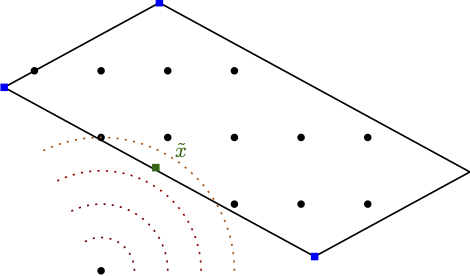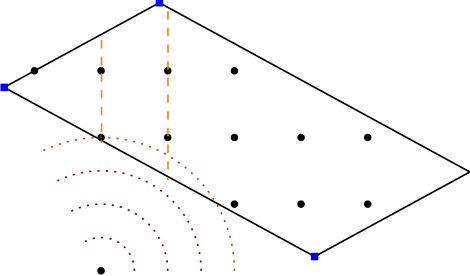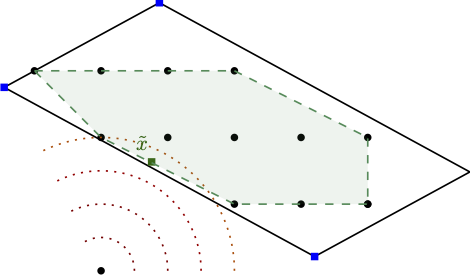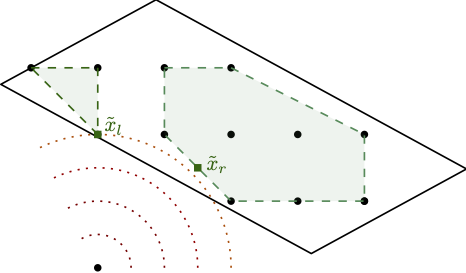Figure 1. Solving stronger subproblems, directly optimizing over the mixed-integer hull can be very powerful. (left) baseline and fractional optimal solution, (middle-left) branching on fractional variable leads only to minor improvement, (middle-right) direct optimization over the mixed-integer hull and fractional solution over the mixed-integer hull, (right) branching once results in optimal solution.

As such, our approach is a Mixed-Integer Conditional Gradient Algorithm that combines a specific Frank-Wolfe algorithm, the Blended Pairwise Conditional Gradient (BPCG) approach of [TTP] (see also the earlier BPCG post), with a branch-and-bound scheme, and solves MIPs as LMOs. Our approach combines several very powerful improvements from conditional gradients with state-of-the-art MIP techniques to obtain a fast algorithm.

### Tricks to make it work

• Leveraging MIP improvements. As our LMOs in the FW subsolver are standards MIPs we can exploit the whole toolbox of MIP solver improvements. In particular, we can use solution pools to collect and reuse previously identified feasible solution. Note, that as our feasible region is never modified (in contrast to, e.g., outer approximation relying on epigraph formulations), all discovered primal solution are globally feasible. In future releases we will also support more advanced reoptimization features of modern MIP solvers, such as carrying over certain presolve information, propagation, and cutting planes.
• Incomplete resolution of nodes. We do not have to solve nodes to near-optimality but rather we can use an adaptive gap strategy to only partially resolve subproblems, significantly reducing the number of iterations in the FW subsolver.
• Warmstarting. We can warmstart the FW subsolver from the run before branching, further cutting down required iterations. For this we can efficiently (as in: for free) write the parent solution as a convex combination of two distinct solutions valid for the left and the right branch, respectively.
• Lazification and blending. We generalize both the lazification [BPZ] and blending approach [BPTW] to apply to the whole tree allowing to aggressively reuse previously found primal feasible solutions. In particular, no primal feasible solution has to be computed or discovered twice.
• Hybrid branching strategy. Finally, we developed a hybrid branching strategy that can further reduce the number of required nodes; however this is highly dependend on the instance.

Combining and exploiting all these things, this results in having only to solve something like 5-8 LMOs calls per node on average and the longer the run and the deeper the tree, the smaller this number is. Asymptotically the average number of LMO calls is approaching something close to 1 as no MIP-feasible solution is computed twice as mentioned above. For a more complex instance, the overall impact of these tricks is shown in Figure 2 below in terms of the average number of LMO calls per node.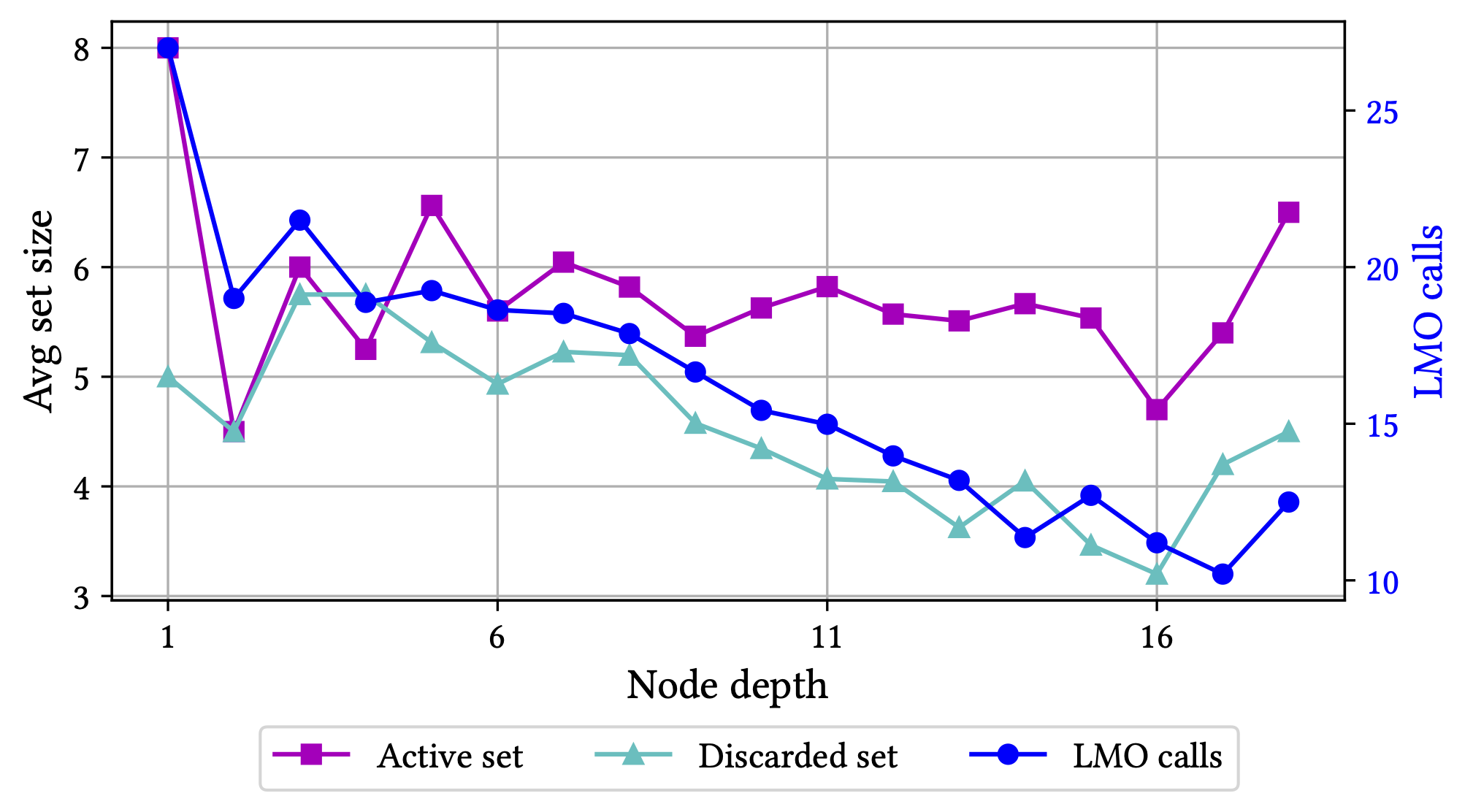Figure 2. Minimizing a quadratic over a cardinality constrained variant of the Birkhoff polytope. Key statistics as a function of the node depth. Both the size of the active (vertex) set and the discarded (vertex) set remain small throughout the run of the algorithm and the average number of LMO calls required to solve a subproblem drops significantly as a function of depth as previously discovered solutions cut out unnecessary LMO calls.

For more details see the preprint [HTBP].

### Implementation of Boscia.jl in a nutshell

• Released under MIT license. Do whatever you want with it and consider contributing to the code base.
• Uses our earlier FrankWolfe.jl Julia package as well the Bonobo.jl branch-and-bound Julia package.
• Uses the Blended Pairwise Conditional Gradient (BPCG) algorithm [TTP] together with (a modified variant of) the adaptive step-size strategy of [PNAJ] from the FrankWolfe.jl package.
• Supports a wide variety of MIP solvers through the MathOptInterface (MOI). Currently, we use SCIP.jl with SCIP 8 in our examples.
• via MOI reads .mps and .lp files out of the box allowing to easily replace linear objectives by convex objectives.
• Interface is identical to that of FrankWolfe.jl: specify the objective and its gradients and provide an LMO for the feasible region.

### Contribute

Most certainly there will be still bugs, calibration issues, and numerical issues in the solver. Any feedback, bug reports, issues, PRs are highly welcome on the package’s github repository.

### A simple example

using Boscia
using FrankWolfe
using Random
using SCIP
using LinearAlgebra
import MathOptInterface
const MOI = MathOptInterface

n = 6

const diffw = 0.5 * ones(n)

##############################
# defining the LMO and using
# SCIP as solver for the LMO
##############################

o = SCIP.Optimizer()

MOI.set(o, MOI.Silent(), true)

for xi in x
end

lmo = FrankWolfe.MathOptLMO(o) # MOI-based LMO

##############################
# defining objective and
##############################

function f(x)
return sum(0.5*(x.-diffw).^2)
end

@. storage = x-diffw
end

##############################
# calling the solver
##############################

x, _, result = Boscia.solve(f, grad!, lmo, verbose = true)


The output - which is quite wide - then roughly looks like this:

Boscia Algorithm.

Parameter settings.
Tree traversal strategy: Move best bound
Branching strategy: Most infeasible
Absolute dual gap tolerance: 1.000000e-06
Relative dual gap tolerance: 1.000000e-02
Frank-Wolfe subproblem tolerance: 1.000000e-05
Total number of varibales: 6
Number of integer variables: 0
Number of binary variables: 6

---------------------------------------------------------------------------------------------------------------------------------------------------------------------------------------------------
Iteration       Open          Bound      Incumbent      Gap (abs)      Gap (rel)       Time (s)      Nodes/sec        FW (ms)       LMO (ms)  LMO (calls c)   FW (Its)   #ActiveSet  Discarded
---------------------------------------------------------------------------------------------------------------------------------------------------------------------------------------------------
*          1          2  -1.202020e-06   7.500000e-01   7.500012e-01            Inf   3.870000e-01   7.751938e+00            237              2              9         13            1          0
100         27   6.249998e-01   7.500000e-01   1.250002e-01   2.000004e-01   5.590000e-01   2.271914e+02              0              0            641          0            1          0
127          0   7.500000e-01   7.500000e-01   0.000000e+00   0.000000e+00   5.770000e-01   2.201040e+02              0              0            695          0            1          0
---------------------------------------------------------------------------------------------------------------------------------------------------------------------------------------------------

Postprocessing

MEMORY_MODE: FrankWolfe.InplaceEmphasis() STEPSIZE: Adaptive EPSILON: 1.0e-7 MAXITERATION: 10000 TYPE: Float64
GRADIENTTYPE: Nothing LAZY: true lazy_tolerance: 2.0
[ Info: In memory_mode memory iterates are written back into x0!

----------------------------------------------------------------------------------------------------------------
Type     Iteration         Primal           Dual       Dual Gap           Time         It/sec     #ActiveSet
----------------------------------------------------------------------------------------------------------------
Last             0   7.500000e-01   7.500000e-01   0.000000e+00   1.086583e-03   0.000000e+00              1
----------------------------------------------------------------------------------------------------------------
PP             0   7.500000e-01   7.500000e-01   0.000000e+00   1.927792e-03   0.000000e+00              1
----------------------------------------------------------------------------------------------------------------

Solution Statistics.
Solution Status: Optimal (tree empty)
Primal Objective: 0.75
Dual Bound: 0.75
Dual Gap (relative): 0.0

Search Statistics.
Total number of nodes processed: 127
Total number of lmo calls: 699
Total time (s): 0.58
LMO calls / sec: 1205.1724137931035
Nodes / sec: 218.96551724137933
LMO calls / node: 5.503937007874016


### References

[TTP] Tsuji, K., Tanaka, K. I., & Pokutta, S. (2021). Sparser kernel herding with pairwise conditional gradients without swap steps. to appear in Proceedings of ICML. arXiv preprint arXiv:2110.12650. pdf

[PNAJ] Pedregosa, F., Negiar, G., Askari, A., & Jaggi, M. (2020, June). Linearly convergent Frank-Wolfe with backtracking line-search. In International Conference on Artificial Intelligence and Statistics (pp. 1-10). PMLR. pdf

[BDRT] Buchheim, C., De Santis, M., Rinaldi, F., & Trieu, L. (2018). A Frank–Wolfe based branch-and-bound algorithm for mean-risk optimization. Journal of Global Optimization, 70(3), 625-644. pdf

[HTBP] Hendrych, D., Troppens, H., Besançon, M., & Pokutta, S. (2022). Convex integer optimization with Frank-Wolfe methods. arXiv preprint arXiv:2208.11010. pdf

[BPTW] Braun, G., Pokutta, S., Tu, D., & Wright, S. (2019, May). Blended conditonal gradients. In International Conference on Machine Learning (pp. 735-743). PMLR. pdf

[BPZ] Braun, G., Pokutta, S., & Zink, D. (2017, July). Lazifying conditional gradient algorithms. In International conference on machine learning (pp. 566-575). PMLR. pdf

]]>
Sebastian Pokutta
Acceleration of Frank-Wolfe algorithms with open loop step-sizes2022-07-05T01:00:00+02:002022-07-05T01:00:00+02:00http://www.pokutta.com/blog/research/2022/07/05/open_loop_fw-abstractTL;DR: This is an informal discussion of our recent paper Acceleration of Frank-Wolfe algorithms with open loop step-sizes by Elias Wirth, Thomas Kerdreux, and Sebastian Pokutta. In the paper, we study accelerated convergence rates for the Frank-Wolfe algorithm (FW) with open loop step-size rules, characterize settings for which FW with open loop step-size rules is non-asymptotically faster than FW with line search or short-step, and provide a partial answer to an open question in kernel herding.

Written by Elias Wirth.

### Introduction and motivation

Frank-Wolfe algorithms (FW) [F], see Algorithm 1, are popular first-order methods to solve convex constrained optimization problems of the form

$\min_{x\in \mathcal{C}} f(x),$

where $\mathcal{C}\subseteq\mathbb{R}^d$ is a compact convex set and $f\colon \mathcal{C} \to \mathbb{R}$ is a convex and smooth function. FW and its variants rely on a linear minimization oracle instead of potentially expensive projection-like oracles. Many works have identified accelerated convergence rates under various structural assumptions on the optimization problem and for specific FW variants when using line search or short-step, requiring feedback from the objective function, see, e.g., [GH13, GH15, GM, J, LJ] for an incomplete list of references.

Algorithm 1. Frank-Wolfe algorith (FW) [F]

Input: Starting point $x_0\in\mathcal{C}$, step-size rule $\eta_t \in [0, 1]$.
$\text{ }$ 1: $\text{ }$ for $t=0$ to $T$ do
$\text{ }$ 2: $\quad$ $p_t\in \mathrm{argmin}_{p\in \mathcal{C}} \langle \nabla f(x_t), p - x_t\rangle$
$\text{ }$ 3: $\quad$ $x_{t+1} \gets (1-\eta_t) x_t + \eta_t p_t$
$\text{ }$ 4: $\text{ }$ end for

However, little is known about accelerated convergence regimes utilizing open loop step-size rules, a.k.a. FW with pre-determined step-sizes, which are algorithmically extremely simple and stable. In our paper, most of the open loop step-size rules are of the form

$\eta_t = \frac{4}{t+4}.$

One of the main motivations for studying FW with open loop step-size rules is an unexplained phenomenon in kernel herding: In the right plot of Figure 3 of [BLO], the authors observe that FW with open loop step-size rules converges at the optimal rate [BLO] of $\mathcal{O}(1/t^2)$, whereas FW with line search or short-step converges at a rate of $\Omega(1/t)$. For this setting, FW with open loop step-size rules converges at the optimal rate, whereas FW with line search or short-step converge at a suboptimal rate. Despite substantial research interest in the connection between FW and kernel herding, so far, this behaviour remained unexplained.

However, kernel herding is not the only problem setting for which FW with open loop step-size rules can converge faster than FW with line search or short-step. In [B], the author proves that when the feasible region is a polytope, the objective function is strongly convex, the optimum lies in the interior of an at least one-dimensional face of the feasible region $\mathcal{C}$, and some other mild assumptions are satisfied, FW with open loop step-size rules asymptotically converges at a rate of $\mathcal{O}(1/t^2)$. Combined with the convergence rate lower bound of $\Omega(1/t^{1+\epsilon})$ for any $\epsilon > 0$ for FW with line search or short-step [W], this characterizes a setting for which FW with open loop step-size rules converges asymptotically faster than FW with line search or short-step.

### Contributions

The main goal of the paper is to address the current gaps in our understanding of FW with open loop step-size rules.

1. Accelerated rates depending on the location of the unconstrained optimum. For FW with open loop step-size rules, the primal gap does not decay monotonously, unlike for FW with line search or short-step. We thus derive a different proof template that captures several of our acceleration results: For FW with open loop step-size rules, we derive convergence rates of up to $\mathcal{O}(1/t^2)$

1. when the feasible region is uniformly convex and the optimum lies in the interior of the feasible region,
2. when the objective satisfies a Hölderian error bound (think, relaxation of strong convexity) and the optimum lies in the exterior of the feasible region,
3. and when the feasible region is uniformly convex and the objective satisfies a Hölderian error bound.

2. FW with open loop step-size rules can be faster than with line search or short-step. We derive a non-asymptotic version of the accelerated convergence result in [B]. More specifically, we show that when the feasible region is a polytope, the optimum lies in the interior of an at least one-dimensional face of $\mathcal{C}$, the objective is stronly convex, and some additional mild assumptions are satisfied, FW with open loop step-size rules converges at a non-asymptotic rate of $O(1/t^2)$. Combined with the convergence rate lower bound for FW with line search or short-step [W], we thus characterize problem instances for which FW with open loop step-size rules converges non-asymptotically faster than FW with line search or short-step.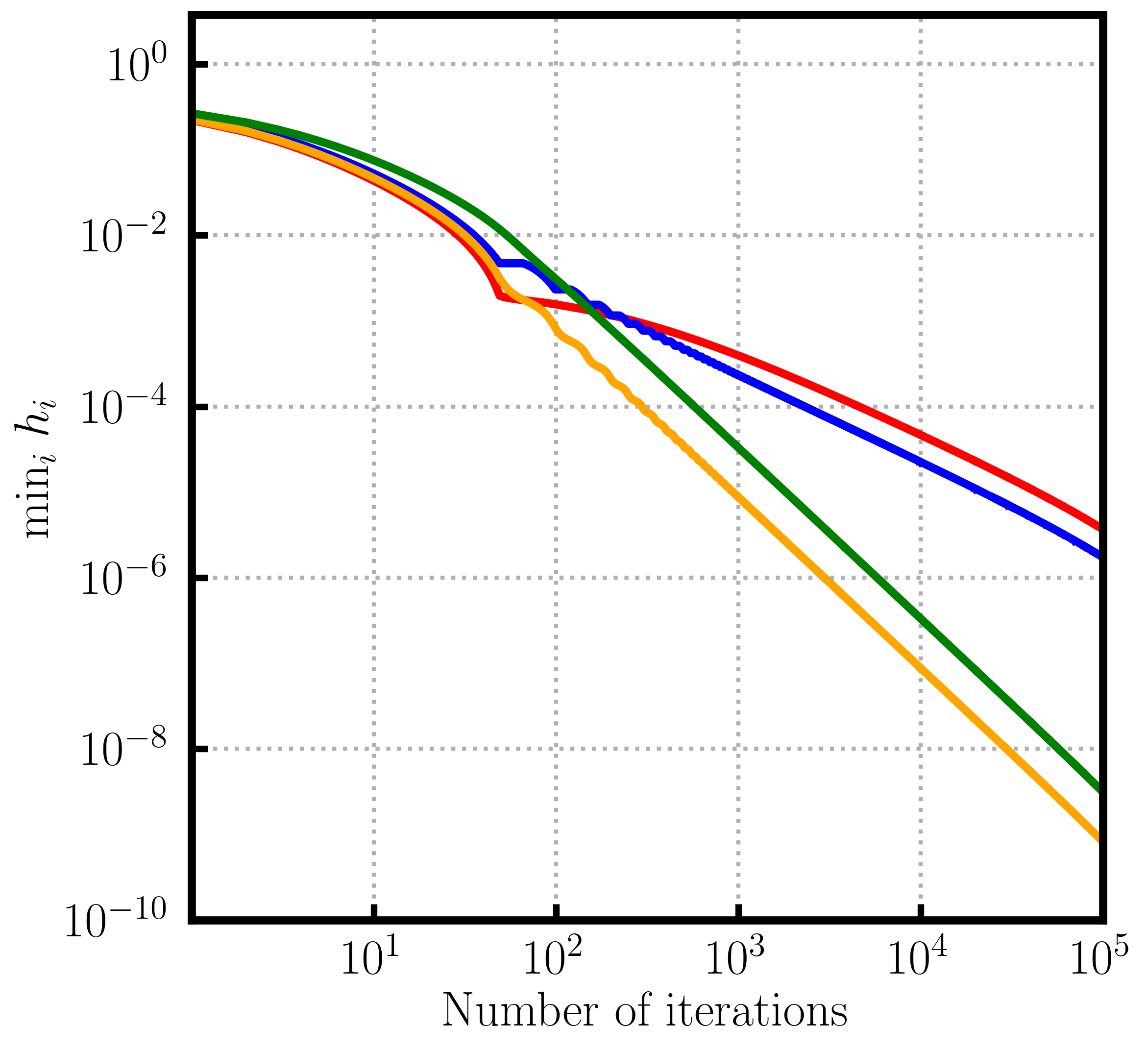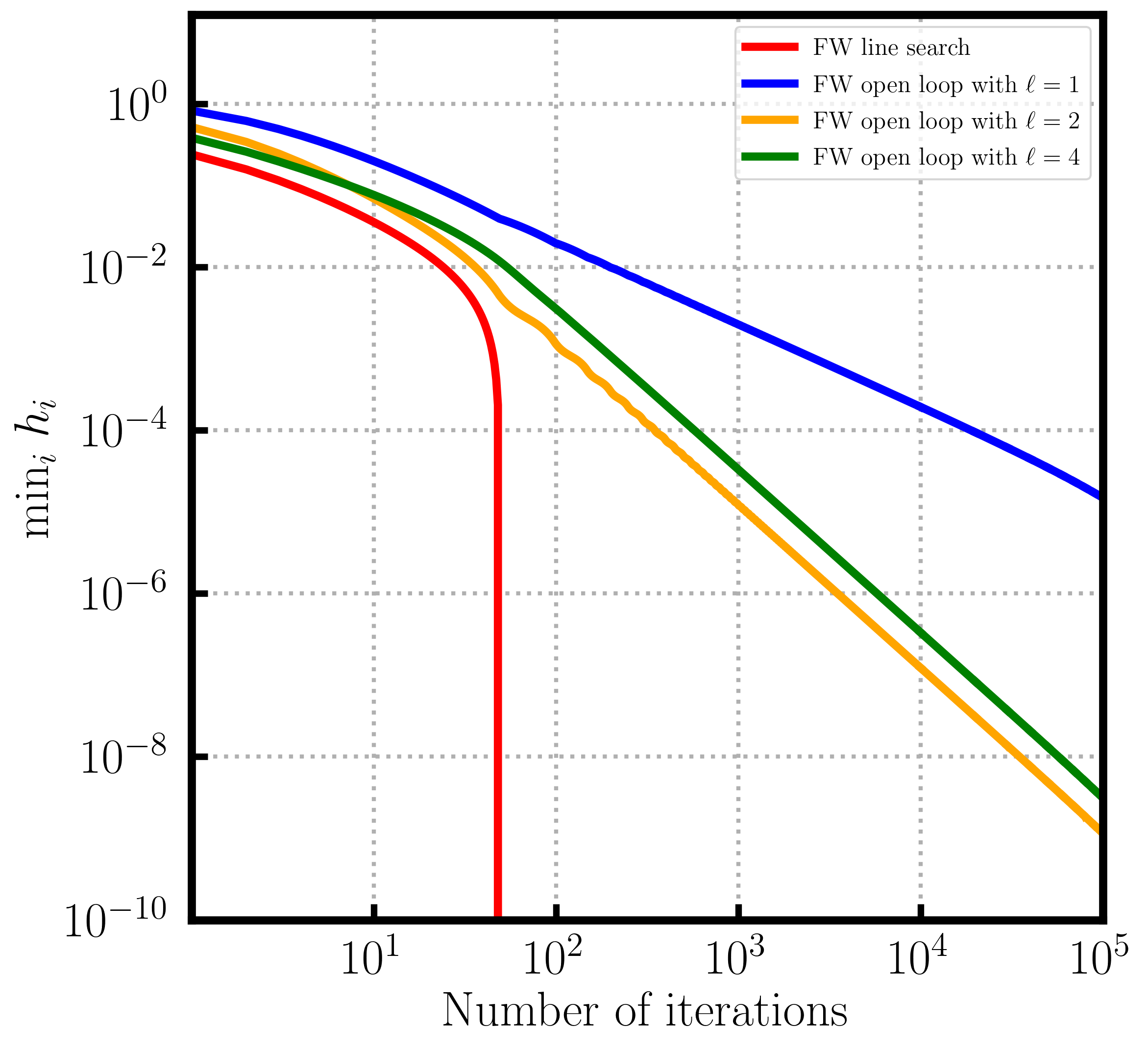Figure 1. Convergence over probability simplex. Depending on the position of the contrained optimum line search (left) can be slower than open loop or (right) faster than open loop.

3. Algorithmic variants. When the feasible region is a polytope, we also study FW variants that were traditionally used to overcome the convergence rate lower bound [W] for FW with line search or short-step. Specifically, we present open loop versions of the Away-Step Frank-Wolfe algorithm (AFW) [LJ] and the Decomposition-Invariant Frank-Wolfe algorithm (DIFW) [GH13]. For both algorithms, we derive convergence rates of order $O(1/t^2)$.

4. Addressing an unexplained phenomenon in kernel herding. We answer the open problem from [BLO], that is, we explain why FW with open loop step-size rules converges at a rate of $\mathcal{O}(1/t^2)$ in the infinite-dimensional kernel herding setting of the right plot of Figure 3 in [BLO].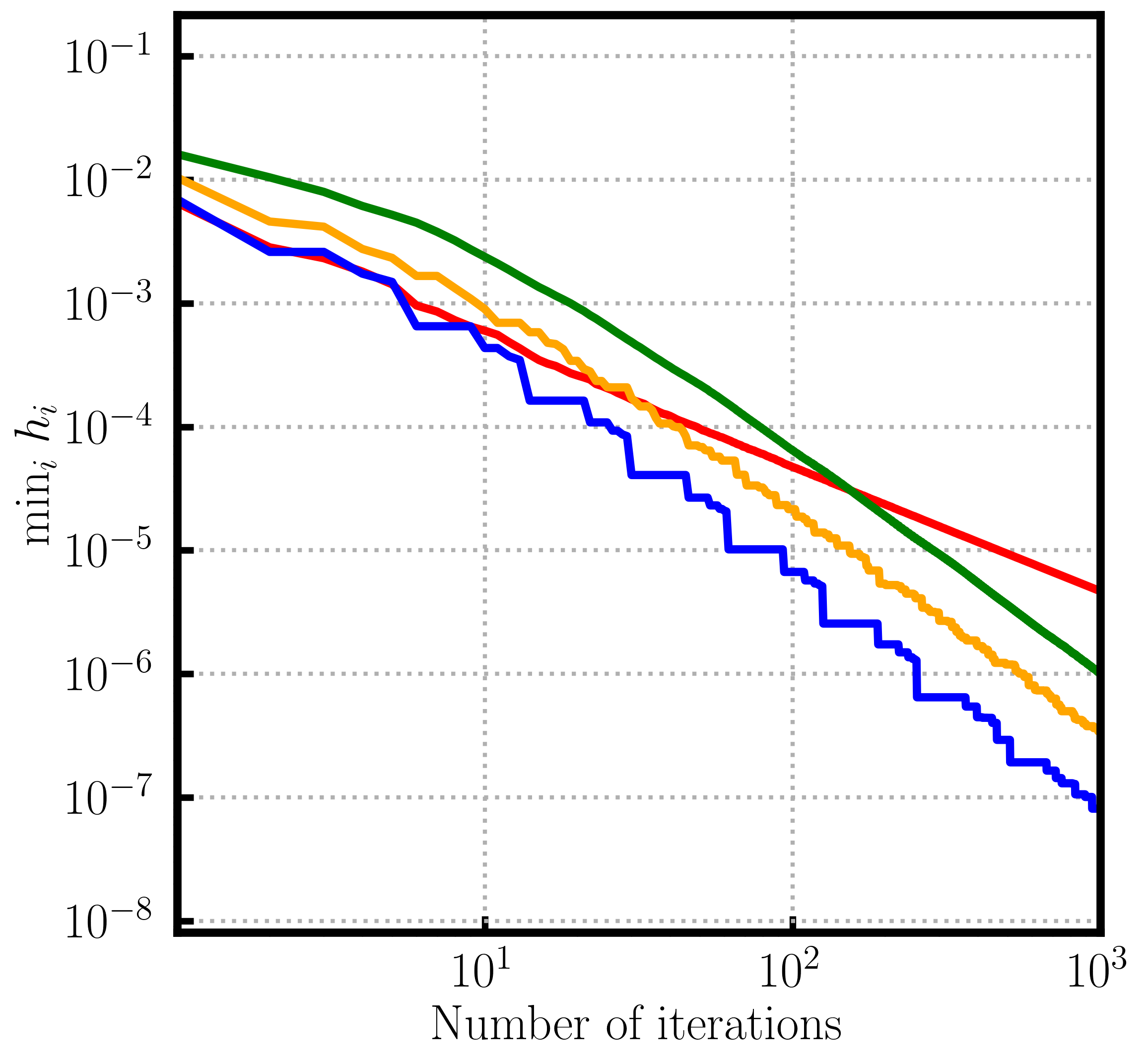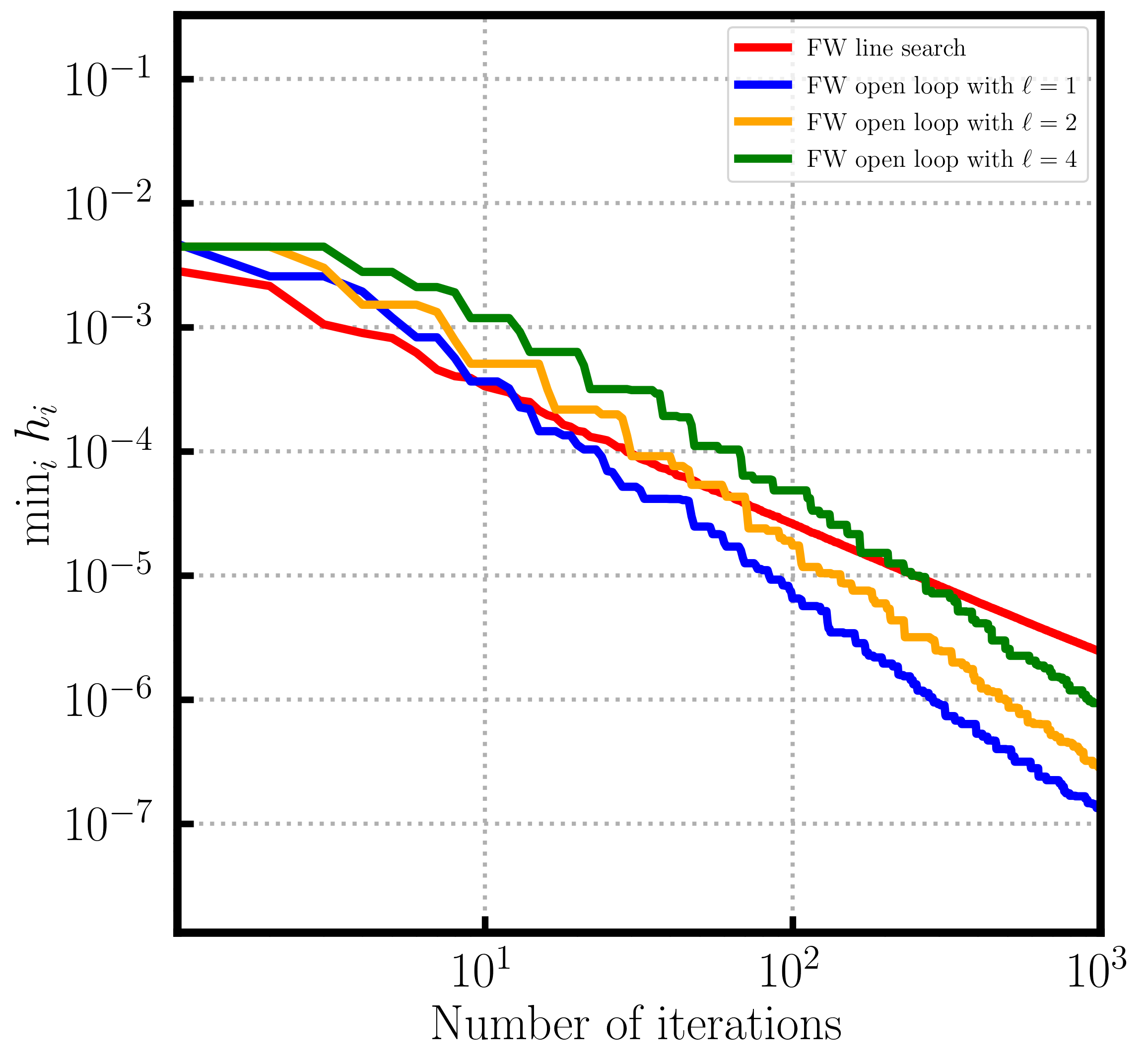Figure 2. Kernel Herding. Open loop step sizes can outperform line search (and short step) (left) uniform (right) non-uniform case.

5. Improved convergence rate after finite burn-in. For many of our results, so as to not contradict the convergence rate lower bound of [J], the derived accelerated convergence rates only hold after an initial number of iterations, that is, the accelerated rates require a burn-in phase. This phenomenon is also referred to as accelerated local convergence [CDLP, DCP]. We study this behvaviour both in theory and with numerical experiments for FW with open loop step-size rules.

### References

[B] Bach, F. (2021). On the effectiveness of richardson extrapolation in data science. SIAM Journal on Mathematics of Data Science, 3(4):1251–1277.

[BLO] Bach, F., Lacoste-Julien, S., and Obozinski, G. (2012). On the equivalence between herding and conditional gradient algorithms. In ICML 2012 International Conference on Machine Learning.

[CDLP] Carderera, A., Diakonikolas, J., Lin, C. Y., and Pokutta, S. (2021a). Parameter-free locally accelerated conditional gradients. arXiv preprint arXiv:2102.06806.

[DCP] Diakonikolas, J., Carderera, A., and Pokutta, S. (2020). Locally accelerated conditional gradients. In International Conference on Artificial Intelligence and Statistics, pages 1737–1747. PMLR.

[F] Frank, M., Wolfe, P., et al. (1956). An algorithm for quadratic programming. Naval research logistics quarterly, 3(1-2):95–110

[GH13] Garber, D. and Hazan, E. (2013). A linearly convergent conditional gradient algorithm with applications to online and stochastic optimization. arXiv preprint arXiv:1301.4666.

[GH15] Garber, D. and Hazan, E. (2015). Faster rates for the frank-wolfe method over strongly-convex sets. In International Conference on Machine Learning, pages 541–549. PMLR.

[GM] Guélat, J. and Marcotte, P. (1986). Some comments on wolfe’s ‘away step’. Mathematical Programming, 35(1):110–119.

[J] Jaggi, M. (2013). Revisiting frank-wolfe: Projection-free sparse convex optimization. In International Conference on Machine Learning, pages 427–435. PMLR.

[LJ] Lacoste-Julien, S. and Jaggi, M. (2015). On the global linear convergence of frank-wolfe optimization variants. Advances in Neural Information Processing Systems, 28:496–504.

[W] Wolfe, P. (1970). Convergence theory in nonlinear programming. Integer and nonlinear programming, pages 1–36.

]]>
Elias Wirth
Pairwise Conditional Gradients without Swap Steps2022-05-21T07:00:00+02:002022-05-21T07:00:00+02:00http://www.pokutta.com/blog/research/2022/05/21/bpcg-abstractTL;DR: This is an informal summary of our recent article Sparser Kernel Herding with Pairwise Conditional Gradients without Swap Steps by Kazuma Tsuji, Ken’ichiro Tanaka, and Sebastian Pokutta, which was accepted at ICML 2022. In this article we present a modification of the pairwise conditional gradient algorithm that removes the dreaded swap steps. The resulting algorithm can even be applied to infinite dimensional feasible regions and promotes high levels of sparsity, which is useful in applications such as e.g., kernel herding.

Written by Sebastian Pokutta.

## What is the paper about and why you might care

One of the most appealing conditional gradient algorithm is the Pairwise Conditional Gradient (PCG) algorithm introduced in [LJ] as a modification of the Away-step Frank-Wolfe (AFW) algorithm. The Pairwise Conditional Gradient algorithm essentially performs a normal Frank-Wolfe step and an away-step simultaneously. This gives much higher convergence steps in practice, however in the analysis now, so-called swap steps appear, where weight is shifted from an away-vertex to a new Frank-Wolfe vertex. For these steps, we cannot bound the primal progress and additionally, there are potentially lots of these steps, so that the theoretical convegence bounds are much worse than what we observe in practice. In fact its guarantees are worse than the guarantees for the Away-step Frank-Wolfe algorithm, that is almost always outperformed by the Pairwise Conditional Gradient algorithm in practice. Various modifications of PCG had been suggested, e.g., in [RZ] and [MGP] to deal with this issue, however often requiring subprocedures whose costs cannot be easily bounded.

It should also be mentioned that the PCG algorithm is particularly nice in the case of polytopes and almost becomes a combinatorial algorithm as the resulting directions are always formed by direction arising from the line-segment between two vertices and thus there are only finitely many such directions.

## Our results

By borrowing machinery from [BPTW], we show that with a minor modification of the PCG algorithm we can avoid drop steps altogether. In a nutshell, the idea is to limit the pairwise directions to those formed by FW vertices and away-vertices from the current active set and only if those steps are not good enough we perform a normal FW step. This way swap steps cannot appear anymore however we require a key technical lemma that shows that the reduced pairwise steps are still good enough. We call this algorithm the Blended Pairwise Conditional Gradient (BPCG) algorithm; see below.

The resulting algorithm has a theoretical convergence rate that is basically the same as the one for the AFW algorithm (up to small constant factors). In fact, it inherits all convergence proofs that hold for the AFW algorithm. Moreover, it exhibits the same convergence speed as (even often faster than) the original PCG algorithm in practice. The algorithm also works in the infinite dimensional setting, which is not true for the original PCG algorithm due to the dimension dependence arising from the number of swap steps in the convergence rate. The iterates produced by BPCG are very sparse, where sparsity is measured in the number of elements in the convex combinations of the iterates making it very suitable, e.g., for kernel herding.

Blended Pairwise Conditional Gradient Algorithm (BPCG); slightly simplified
Input: Smooth convex function $f$ with first-order oracle access, feasible region (polytope) $P$ with linear optimization oracle access, initial vertex $x_0 \in P$.
Output: Sequence of points $x_0, \dots, x_{T}$
$$S_0 = \{x_0\}$$
For $t = 0, \dots, T-1$ do:
$\quad a_t \leftarrow \arg\max_{v \in S_t} \langle \nabla f(x_{t}), v \rangle$ $\qquad$ {Away-vertex over $S_t$}
$\quad s_t \leftarrow \arg\min_{x \in S_t} \langle \nabla f(x_{t}), v \rangle$ $\qquad$ {Local FW-vertex over $S_t$}
$\quad w_t \leftarrow \arg\min_{x \in P} \langle \nabla f(x_{t}), v \rangle$ $\qquad$ {(Global) FW-vertex over $P$}
$\quad$ If $$\langle \nabla f(x_{t}), a_t - s_t \rangle \geq \langle \nabla f(x_{t}), x_t - w_t \rangle$$: $\qquad$ {Local gap as large as global gap}
$\quad\quad$ $d_t = a_t - s_t$ $\qquad$ {Pick (local) pairwise direction}
$\quad\quad$ $x_{t+1} \leftarrow x_t - \gamma_t d_t$ via line search s.t. residual weight of $a_t$ nonnegative
$\quad\quad$ If $a_t$ removed then {Drop step} $$S_{t+1} \leftarrow S_t \setminus \{a_t\}$$ else {Descent step} $$S_{t+1} \leftarrow S_t$$
$\quad$ Else $\qquad$ {Normal FW Step}
$\quad\quad$ $d_t = x_t - w_t$ $\qquad$ {Pick FW direction}
$\quad\quad$ $x_{t+1} \leftarrow x_t - \gamma_t d_t$ via line search with $\gamma_t \in [0,1]$
$\quad\quad$ Update $$S_{t+1} \leftarrow S_t \cup \{w_t\}$$

The key to the convergence proofs is the following lemma that show that these local pairwise steps combined with global FW steps are good enough to ensure sufficient progress per iteration

Key Lemma. In each iteration $t$ it holds: $2 \langle \nabla f(x_{t}), d_t \rangle \geq \langle \nabla f(x_{t}), a_t - w_t \rangle.$

For those in the know this is all you need to prove convergence: the term on the right-hand side is the strong Wolfe gap and as such progress from smoothness with $d_t$ can be lower bounded by the progress from smoothness with the strong Wolfe gap. See e.g., Cheat Sheet: Smooth Convex Optimization and Cheat Sheet: Linear convergence for Conditional Gradients (towards the end when analyzing AFW) to understand how one continues from here. Also similarly, with this inequality we can apply e.g., the reasoning in Cheat Sheet: Hölder Error Bounds (HEB) for Conditional Gradients to extend the results, to e.g., sharp functions etc.

### Computations

We also performed several computational experiments to evaluate the performance of BPCG and in fact the algorithm has been also implemented in the FrankWolfe.jl Julia Package (See this post or [BCP]) and is now the recommended default active-set based conditional gradient algorithm. With a simple trick by adding a factor on the left-hand side in front of the gap test in the algorithm one can further improve sparsity; see the original paper for more details.

Below in Figure 1, we provide a simple convergence test for the approximate Carathéodory problem. We can see that in iterations BPCG is basically identical to PCG (as expected) however in time it is faster as the local updates are often cheaper.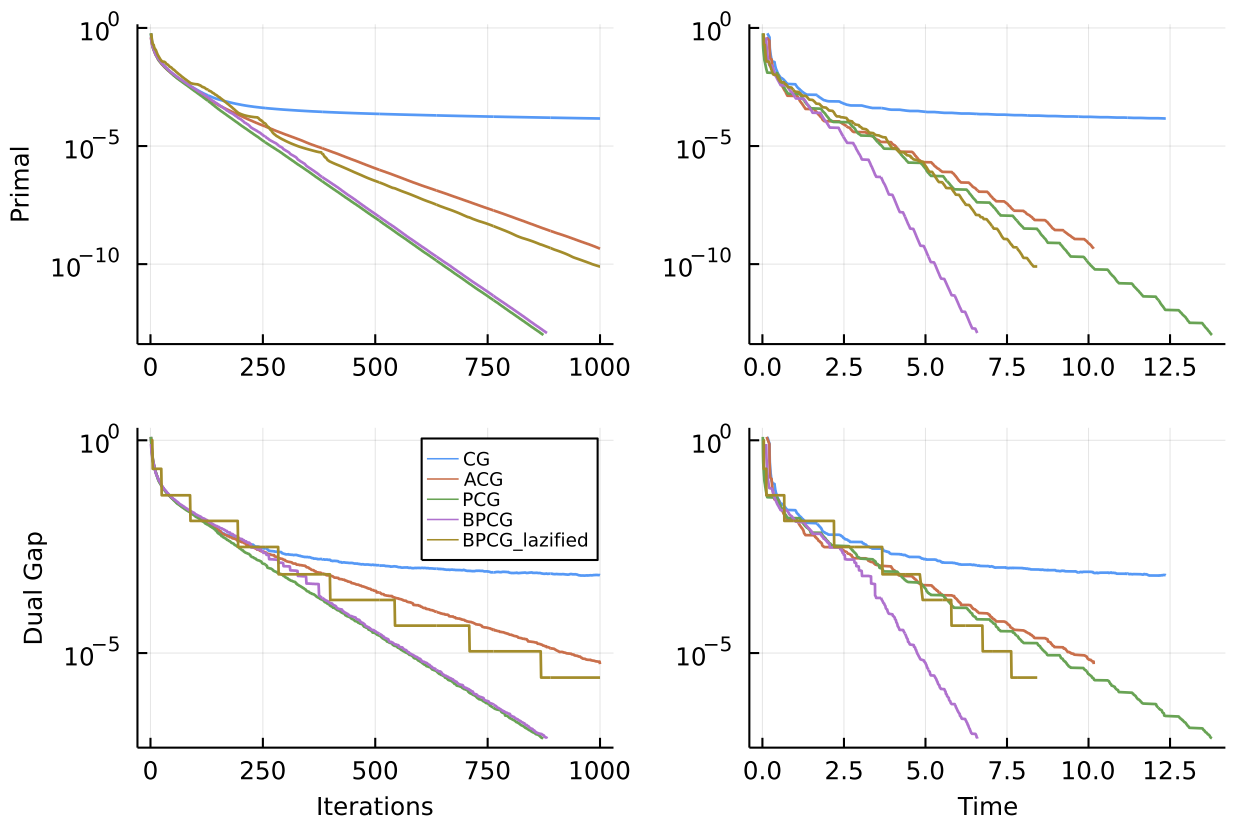Figure 1. Convergence on approximate Carathéodory instance over polytope of dimension $n=200$.

#### Sparsity

While the convergence plot above is quite typical and in terms of speed per iteration or wallclock time BPCG is usually at least as good as PCG (and sometimes faster), the real advantage is often in terms of sparsity as the preference for local steps promotes sparsity. This can be seen in the two plots below.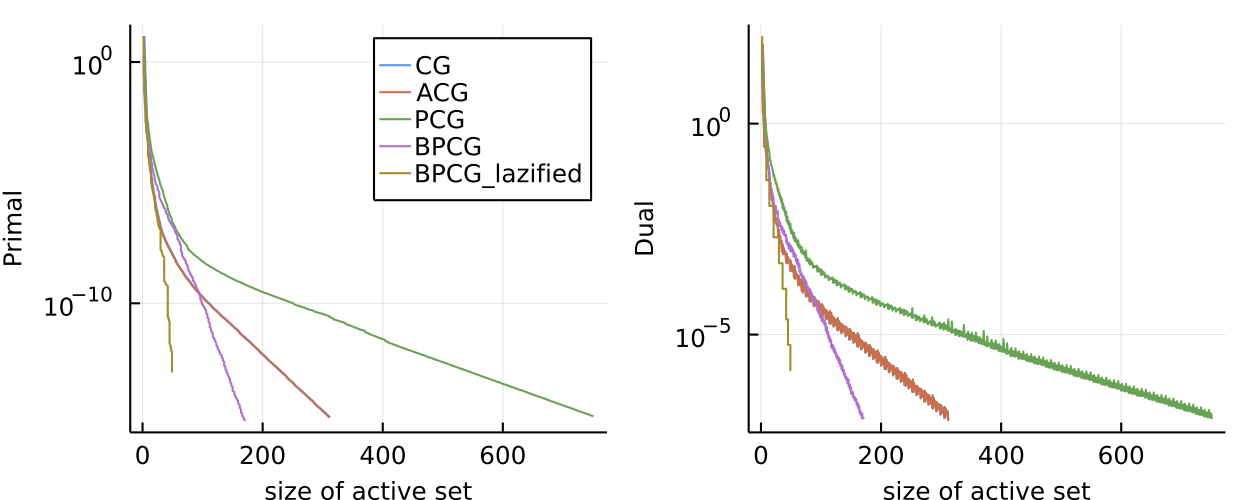Figure 2. Sparse regression problem over $l_5$-norm ball. Here we plot primal value and dual gap vs. size of the active set. BPCG consistently delivers smaller primal and dual values for the same number of atoms in the active set.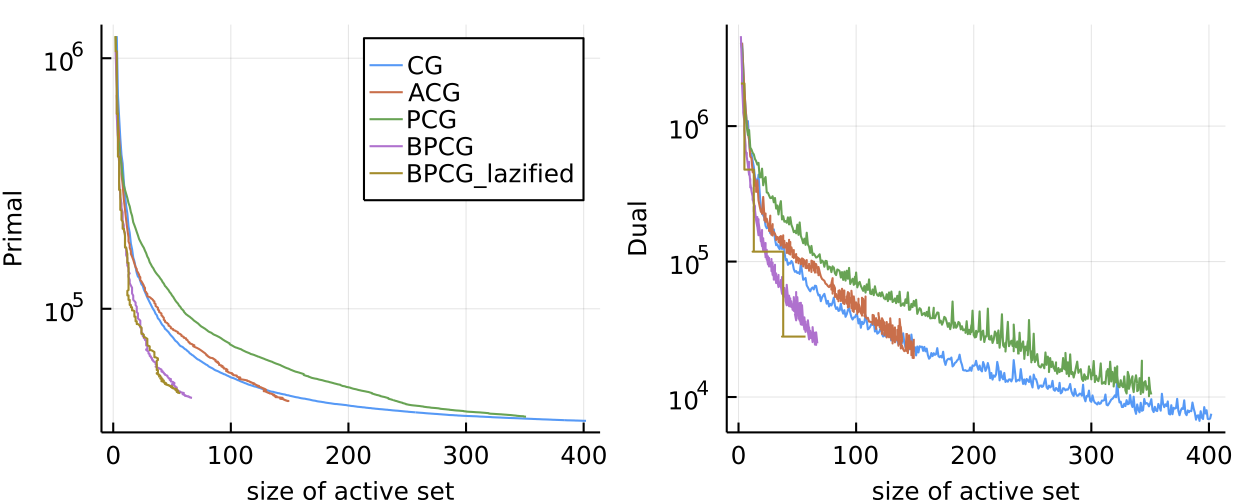Figure 3. Movielens matrix completion problem. Same logic as above with similar results.

#### Kernel Herding

Finally we also considered various kernel herding problems. Here are two examples; the graphs are a little packed.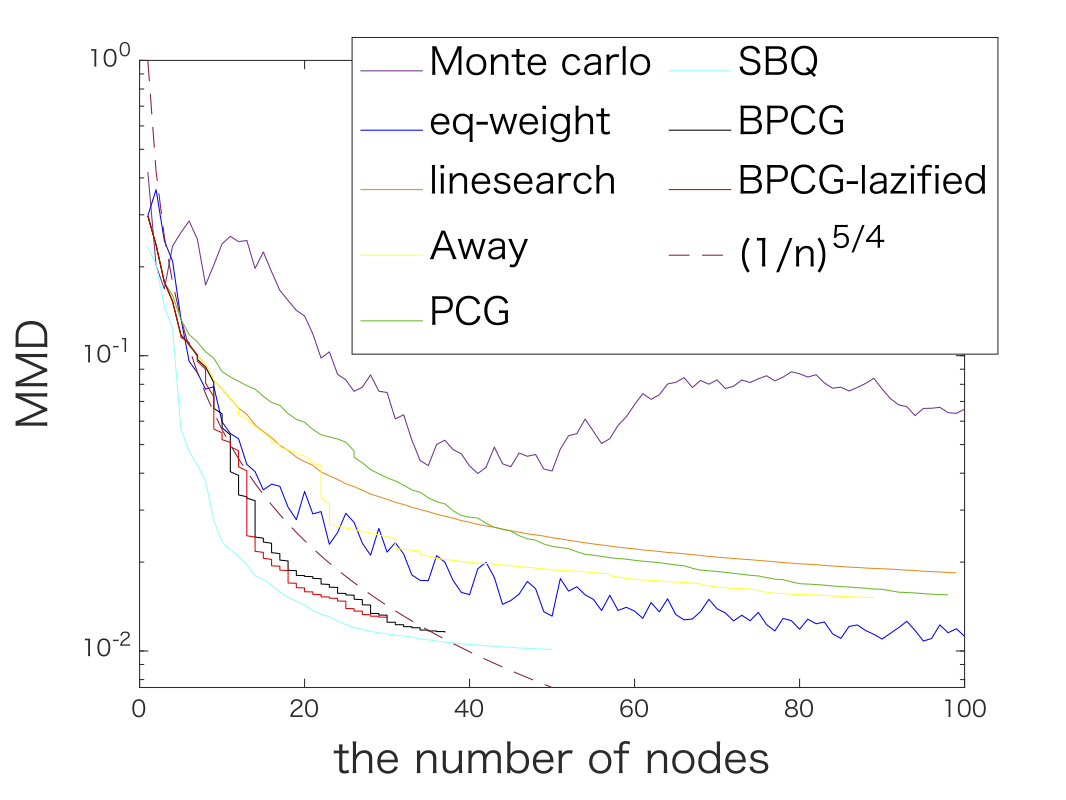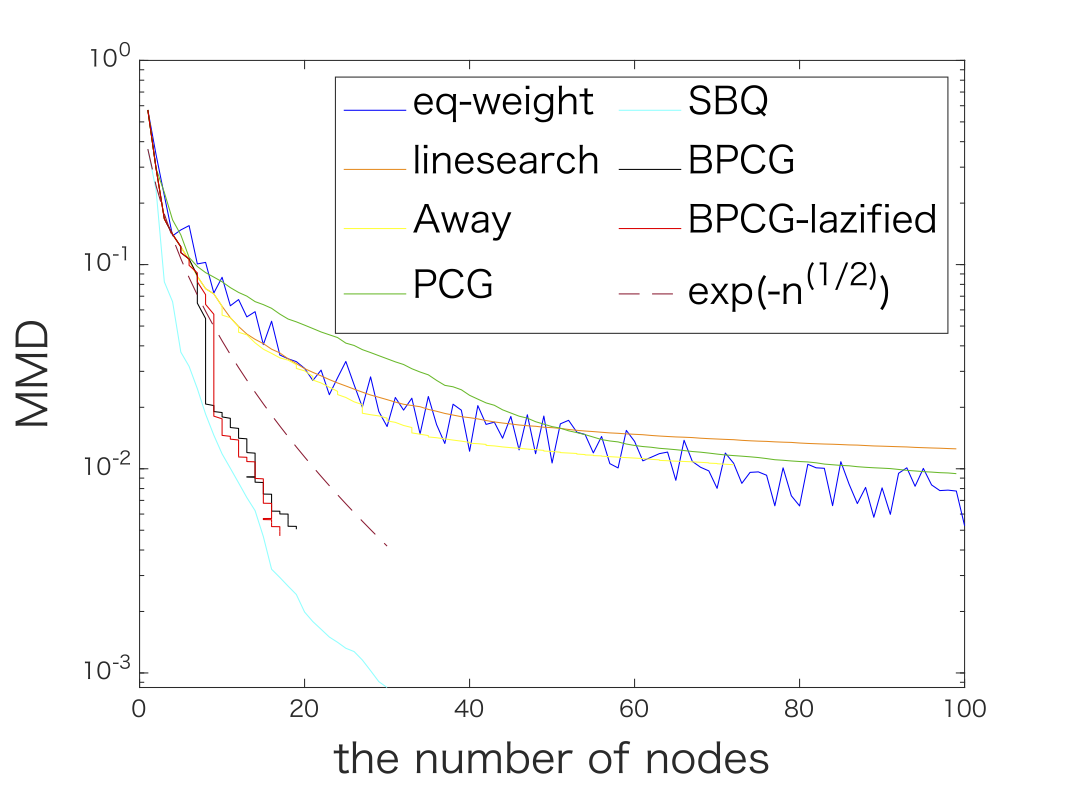Figure 4. Kernel herding for Matérn kernel (left) and Gaussian kernel (right). In both cases BPCG delivers results on par with the Sequential Bayesian Quadrature method (SBQ) however at the fraction of the cost.

### References

[LJ] Lacoste-Julien, S., & Jaggi, M. (2015). On the global linear convergence of Frank-Wolfe optimization variants. In Advances in Neural Information Processing Systems (pp. 496-504). pdf

[RZ] Rinaldi, F., & Zeffiro, D. (2020). A unifying framework for the analysis of projection-free first-order methods under a sufficient slope condition. arXiv preprint arXiv:2008.09781. pdf

[MGP] Mortagy, H., Gupta, S., & Pokutta, S. (2020). Walking in the shadow: A new perspective on descent directions for constrained minimization. Advances in Neural Information Processing Systems, 33, 12873-12883. pdf

[BPTW] Braun, G., Pokutta, S., Tu, D., & Wright, S. (2019, May). Blended conditonal gradients. In International Conference on Machine Learning (pp. 735-743). PMLR. pdf

[BCP] Besançon, M., Carderera, A., & Pokutta, S. (2022). FrankWolfe. jl: A High-Performance and Flexible Toolbox for Frank–Wolfe Algorithms and Conditional Gradients. INFORMS Journal on Computing. pdf

]]>
Sebastian Pokutta